# RD Sharma Solutions for Class 11 Maths Chapter 15 Linear Inequations

## RD Sharma Solutions Class 11 Maths Chapter 15 – Free PDF Download

RD Sharma Solutions for Class 11 Maths Chapter 15 – Linear Inequations are given here for students to study and secure good marks in the main examination. In this chapter, we will study linear inequations in one and two variables. The knowledge of linear inequations is very helpful in solving problems in science, mathematics, Engineering, Linear programming etc.

Expert tutors at BYJU’S have designed these RD Sharma Solutions in a very lucid manner that helps students solve problems in the most efficient possible ways. The solution module also utilizes various shortcut tips and practical examples to explain all the exercise questions in a simple and easily understandable language. Students who aim to score high marks can refer to RD Sharma Class 11 Maths Solutions pdf, from the links given below.

Chapter 15 – Linear Inequations contains six exercises and the RD Sharma Solutions present in this page provide solutions to the questions present in each exercise. Now, let us have a look at the concepts discussed in this chapter.

• Solutions of an inequation.
• Solving linear inequations in one variable.
• The solution of a system of linear inequations in one variable.
• Some applications of linear inequations in one variable.
• Graphical solution of linear inequations in two variables.
• The solution of simultaneous linear inequations in two variables.

## Download the pdf of RD Sharma Solutions for Class 11 Maths Chapter 15 – Linear Inequations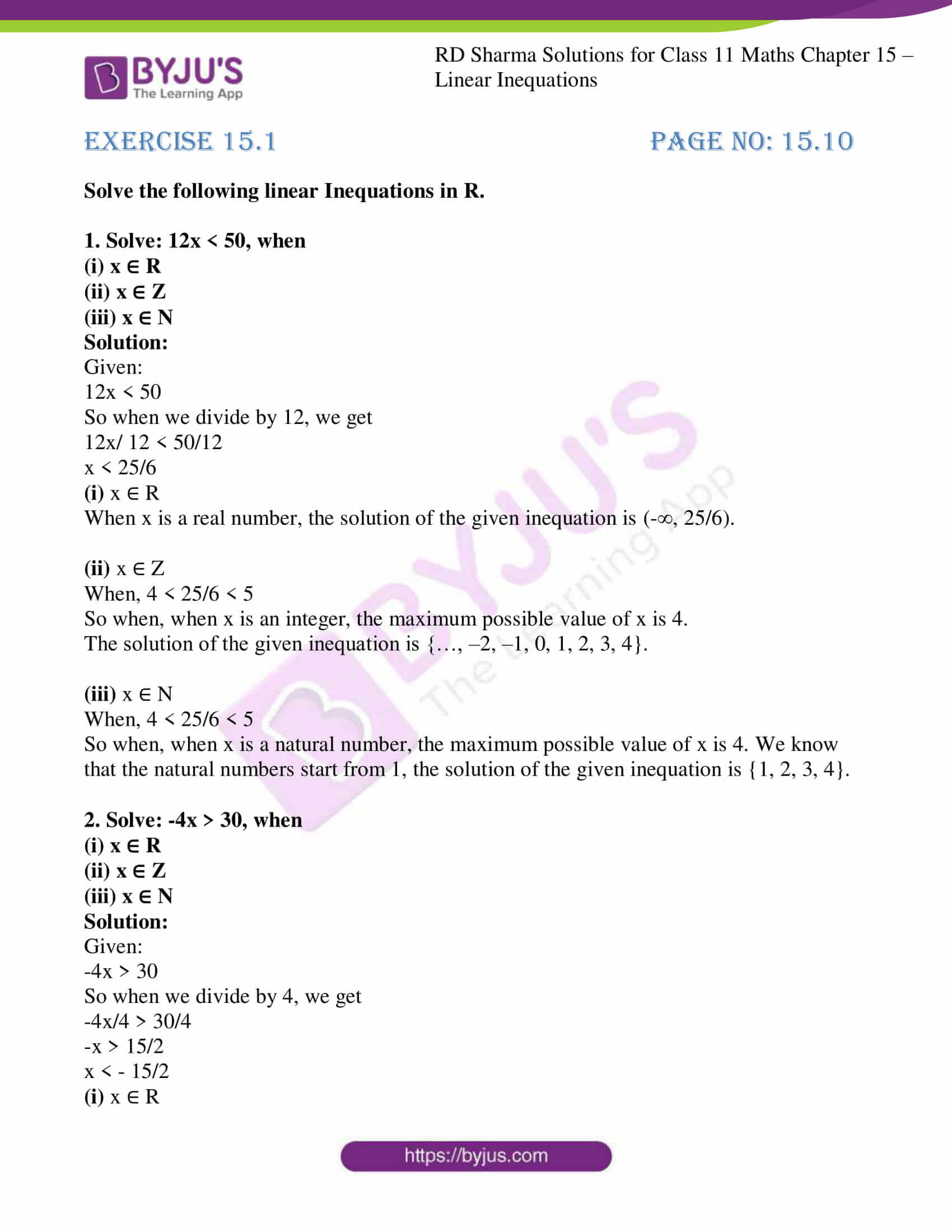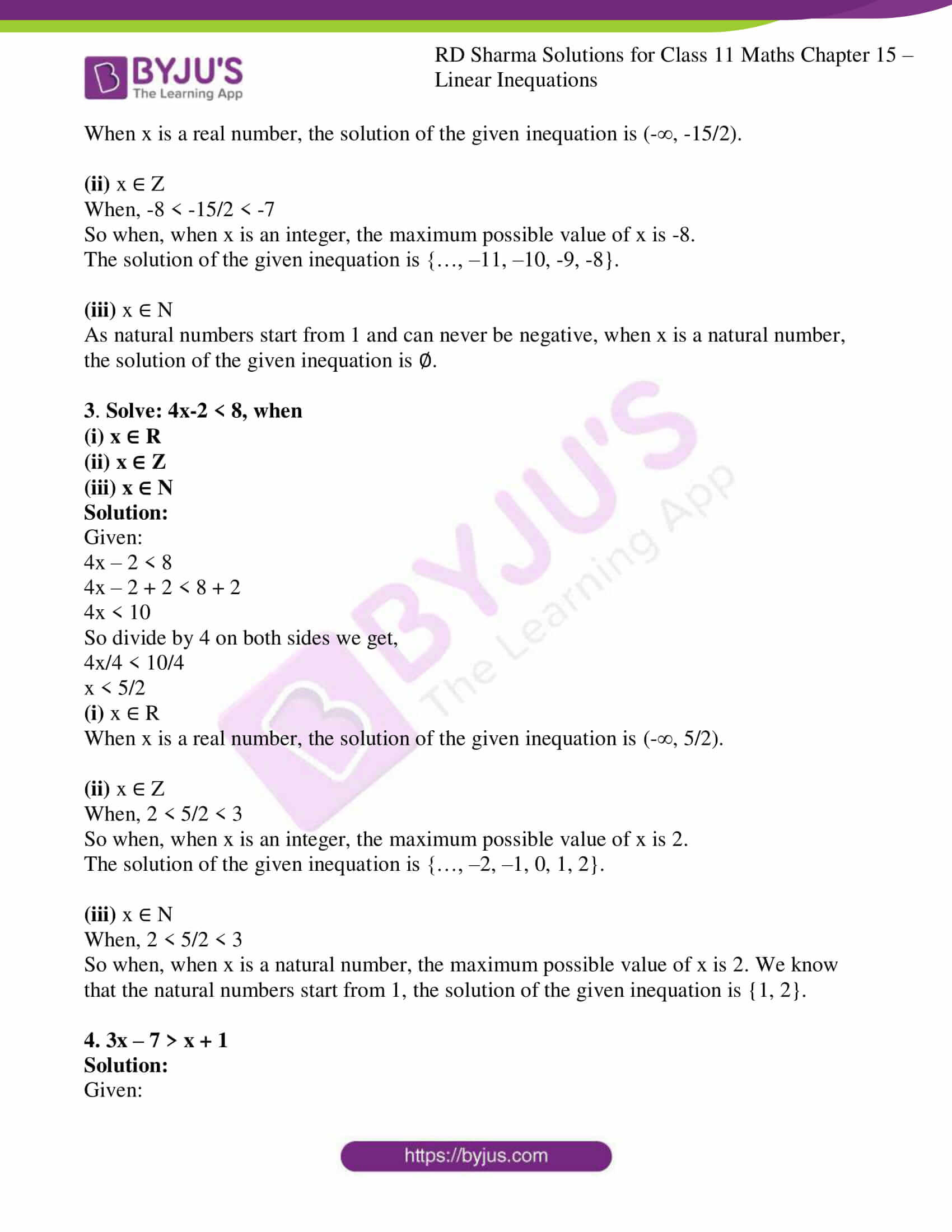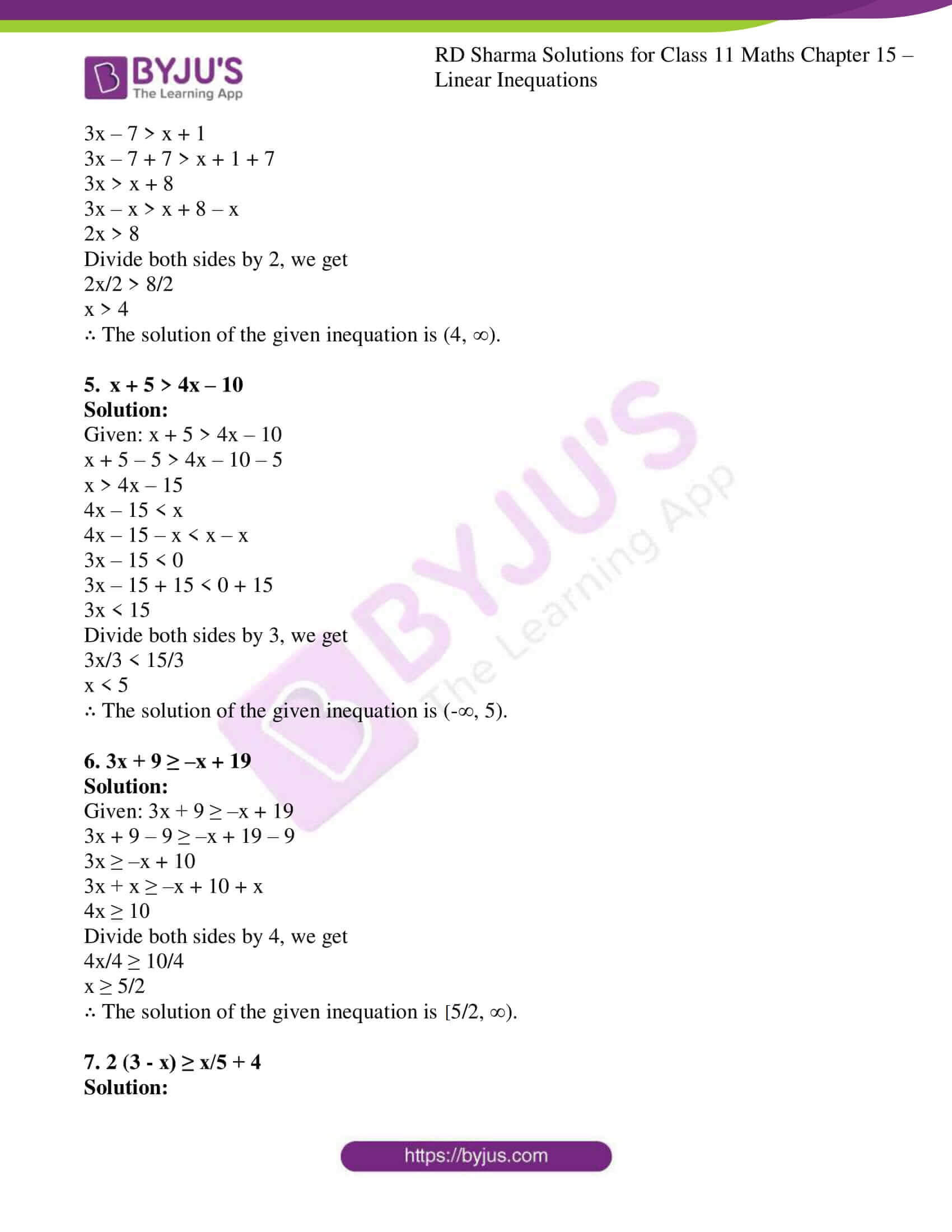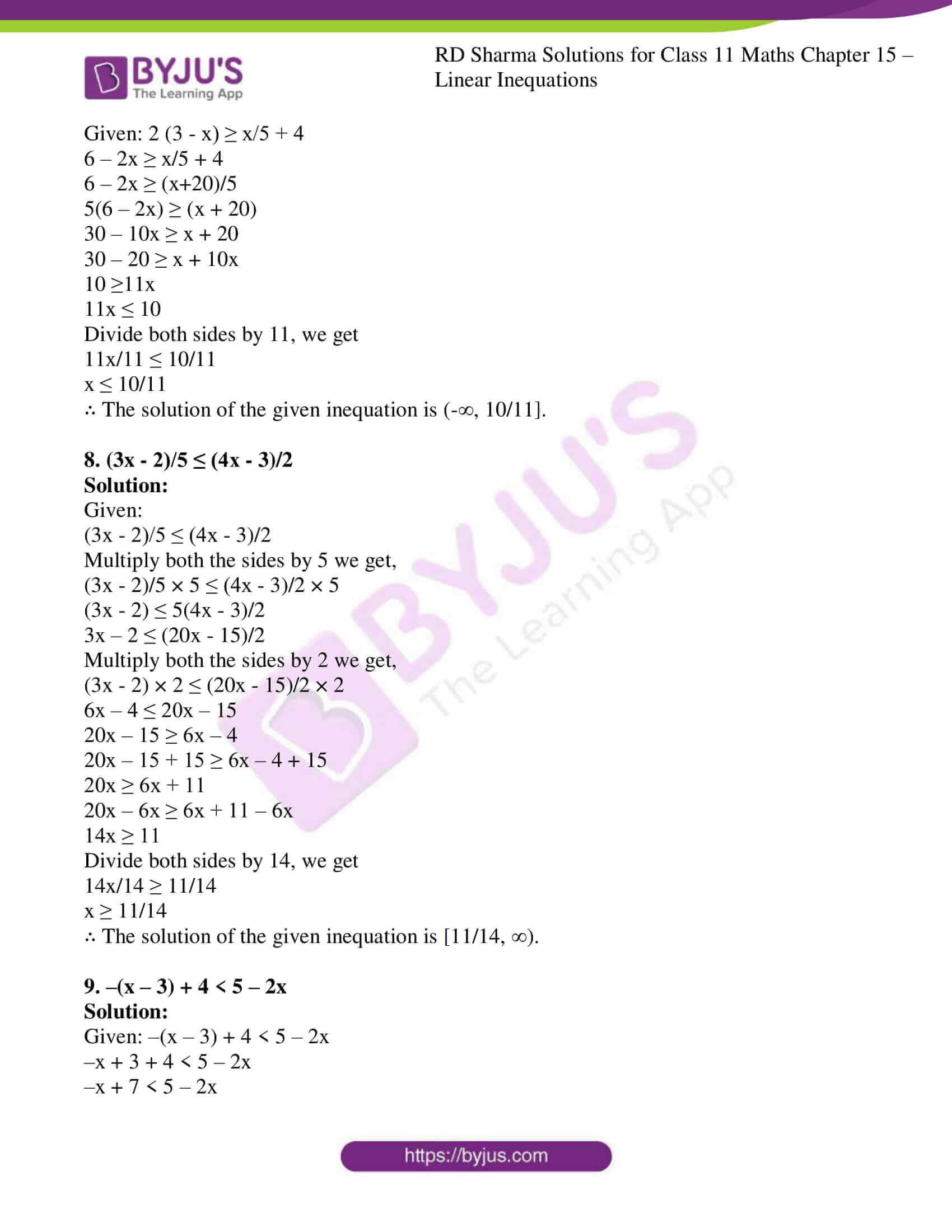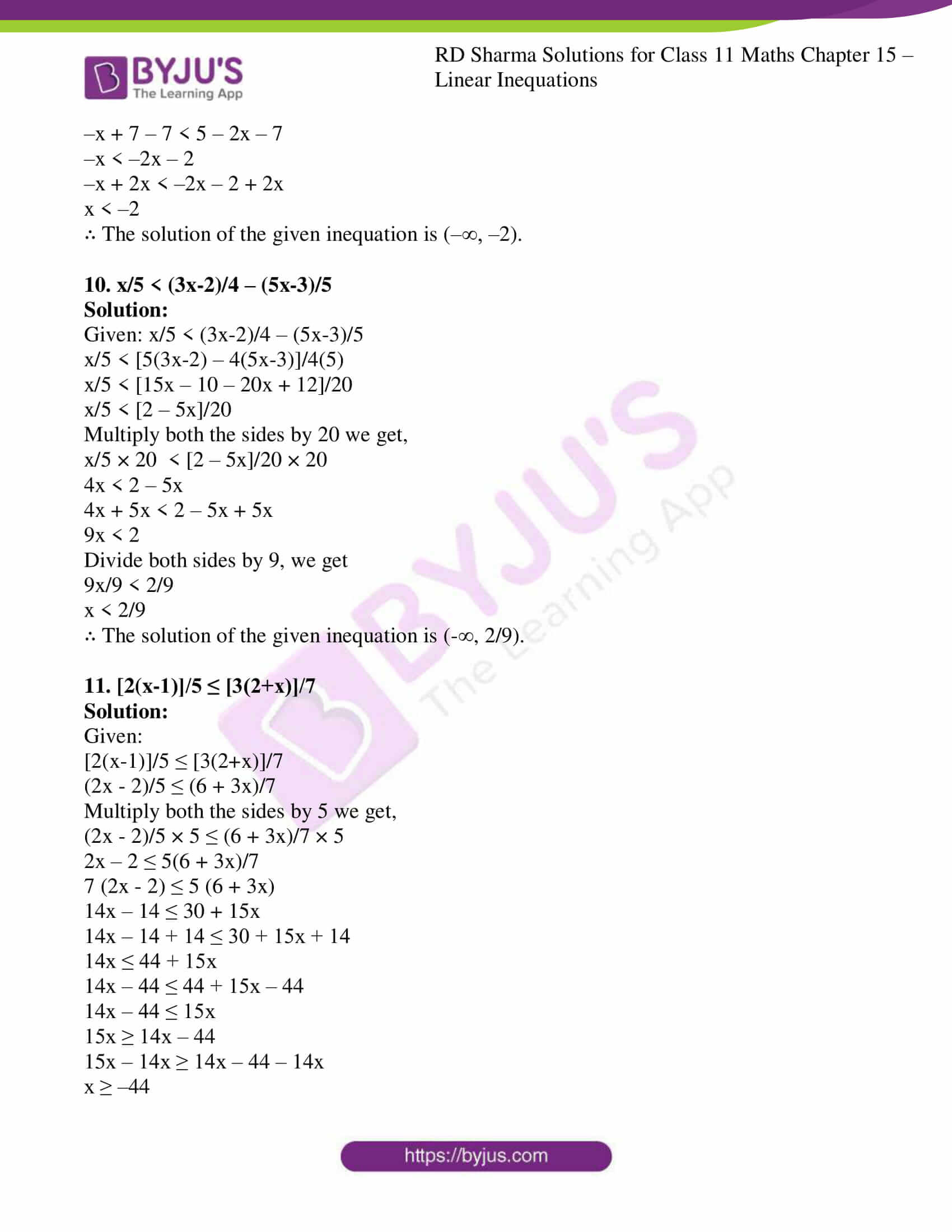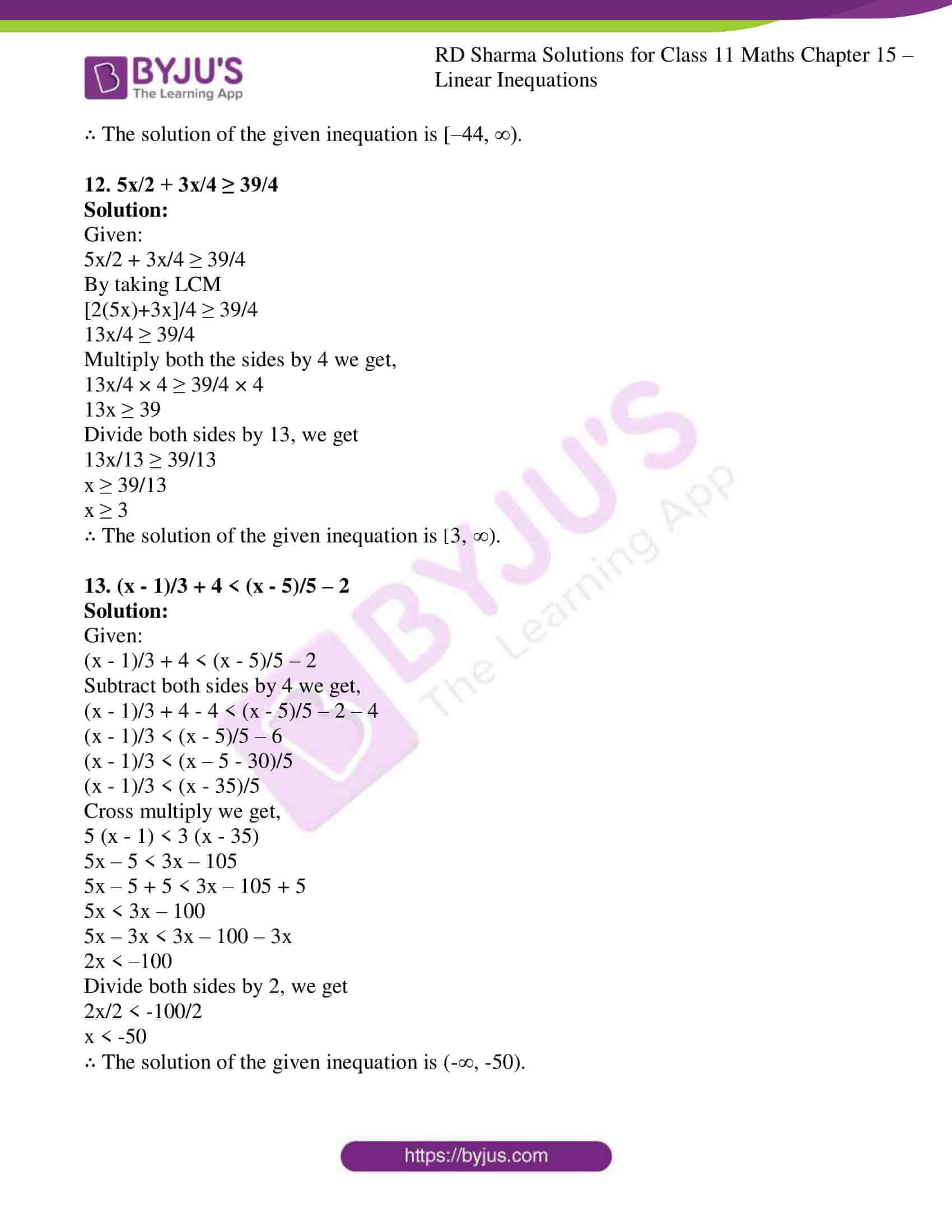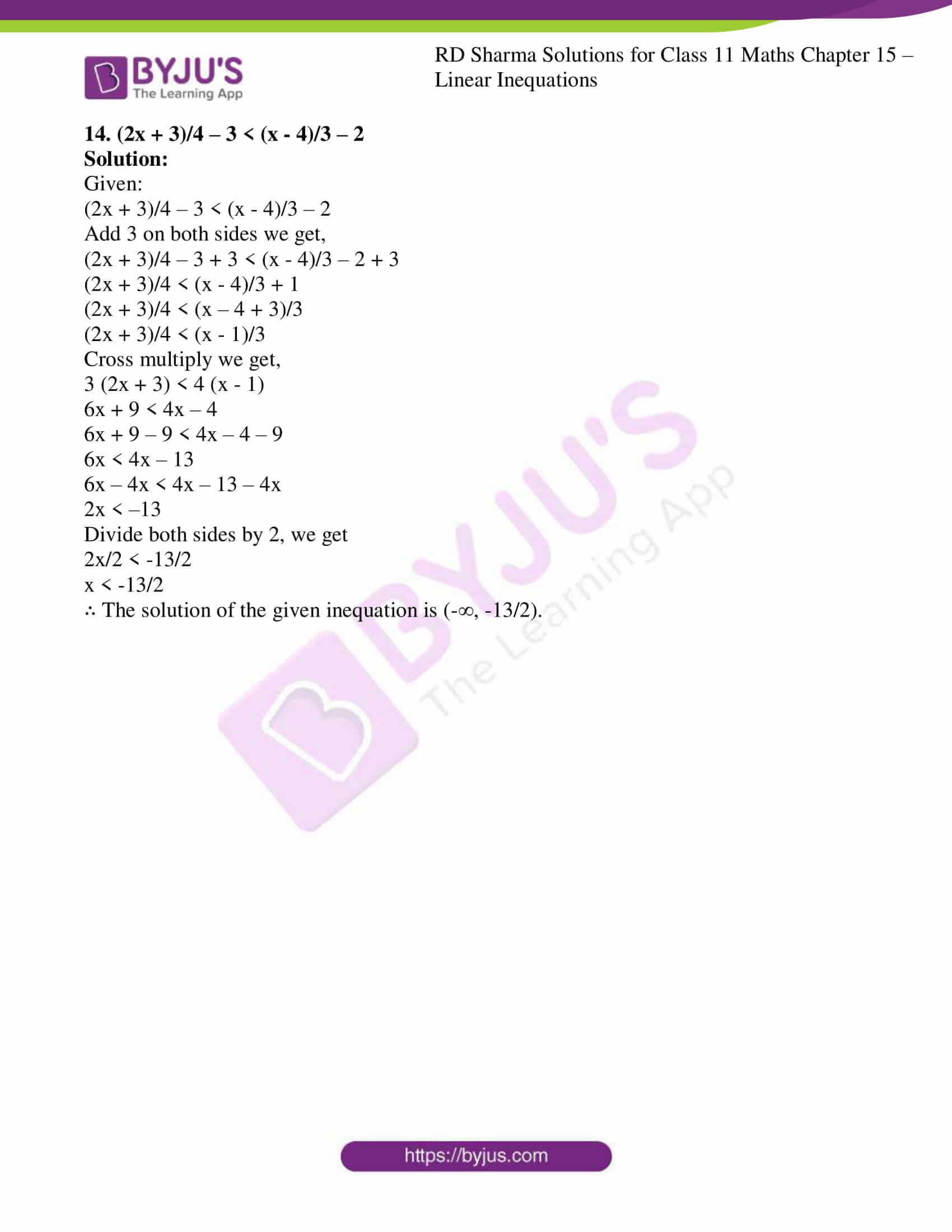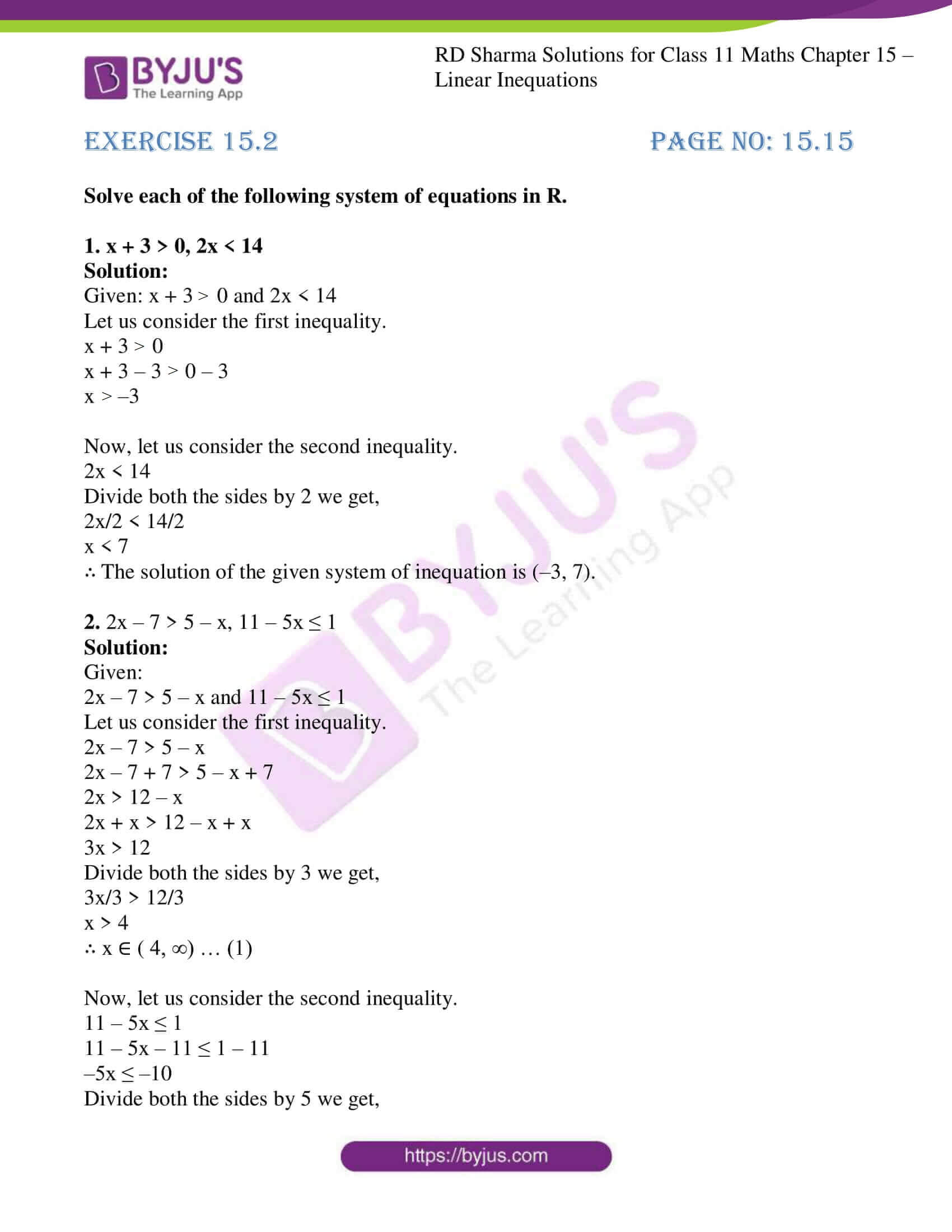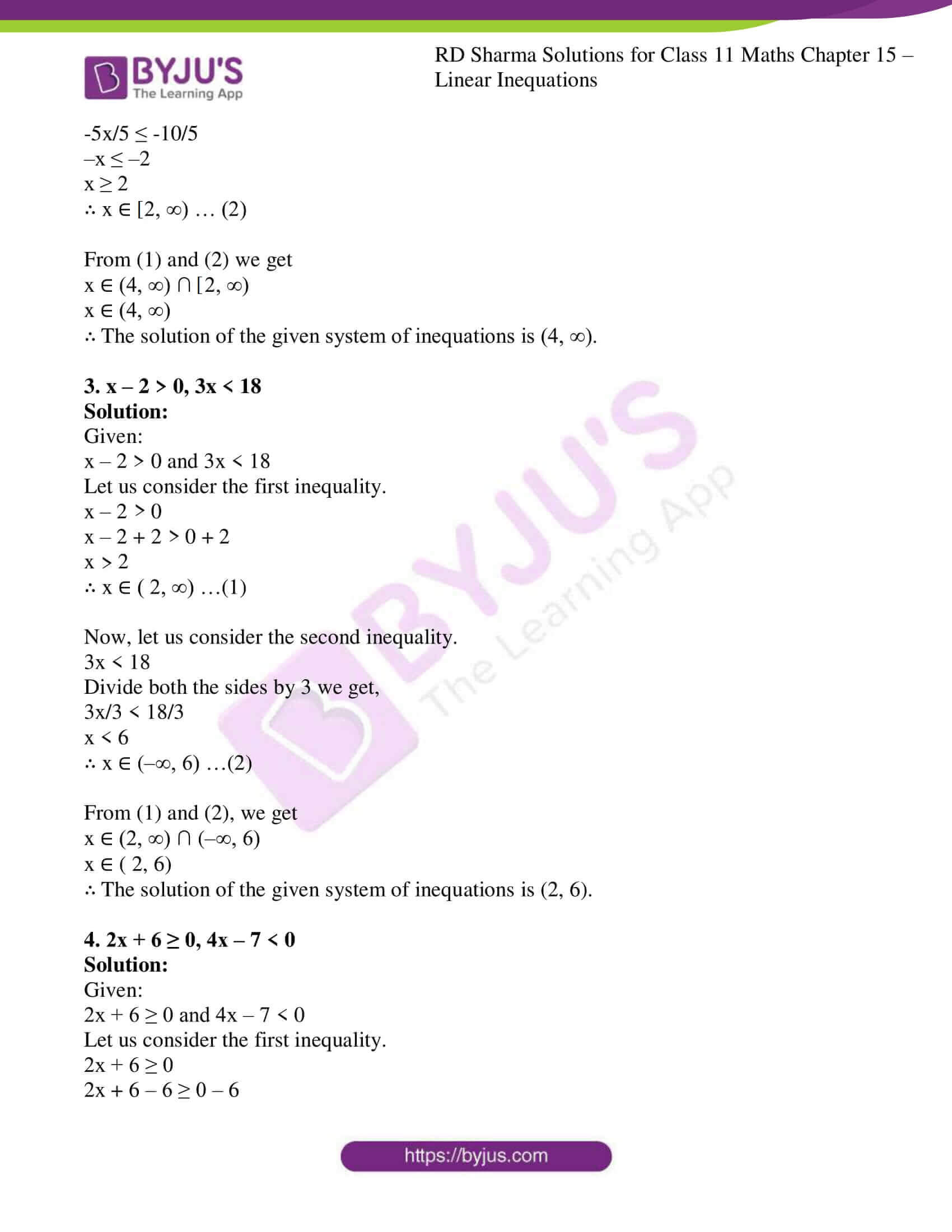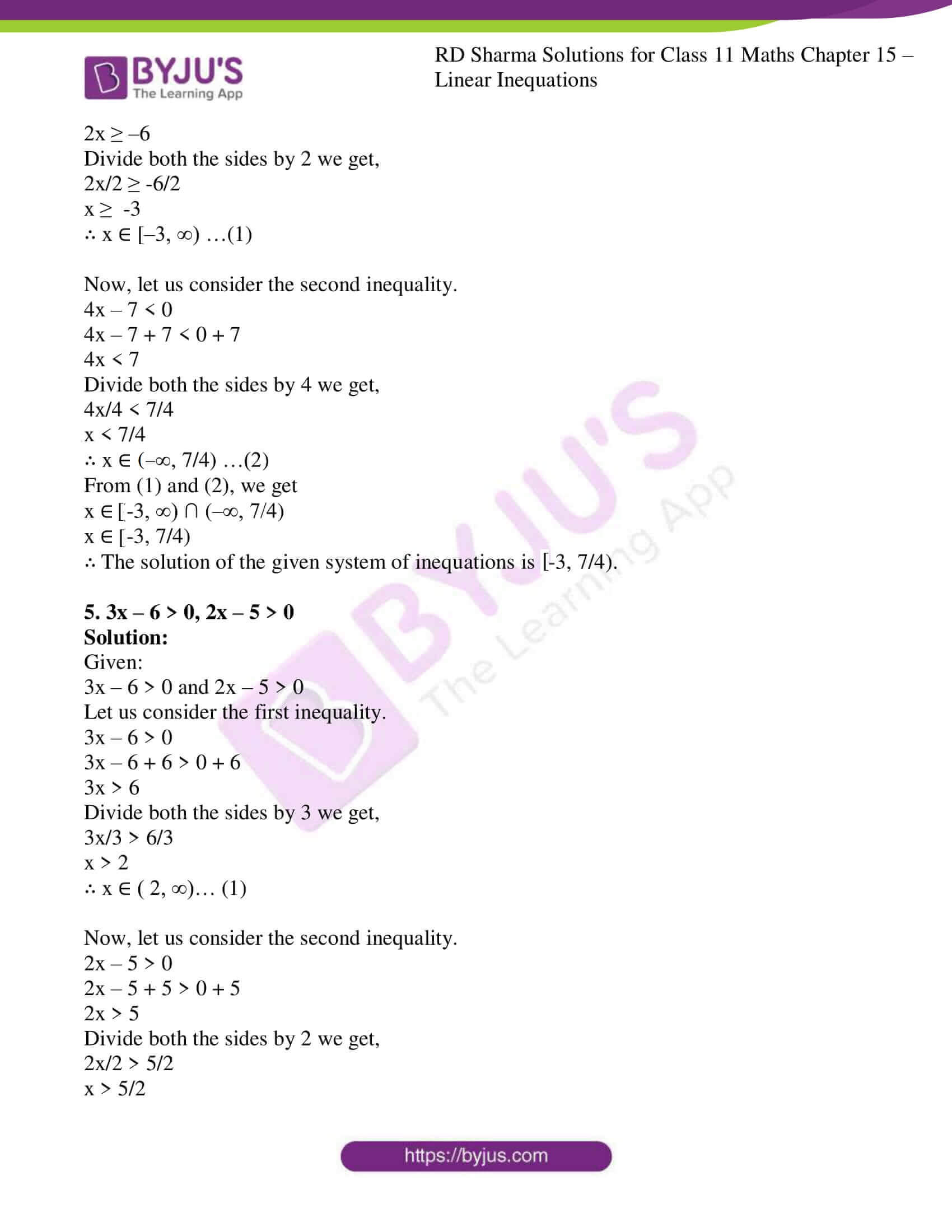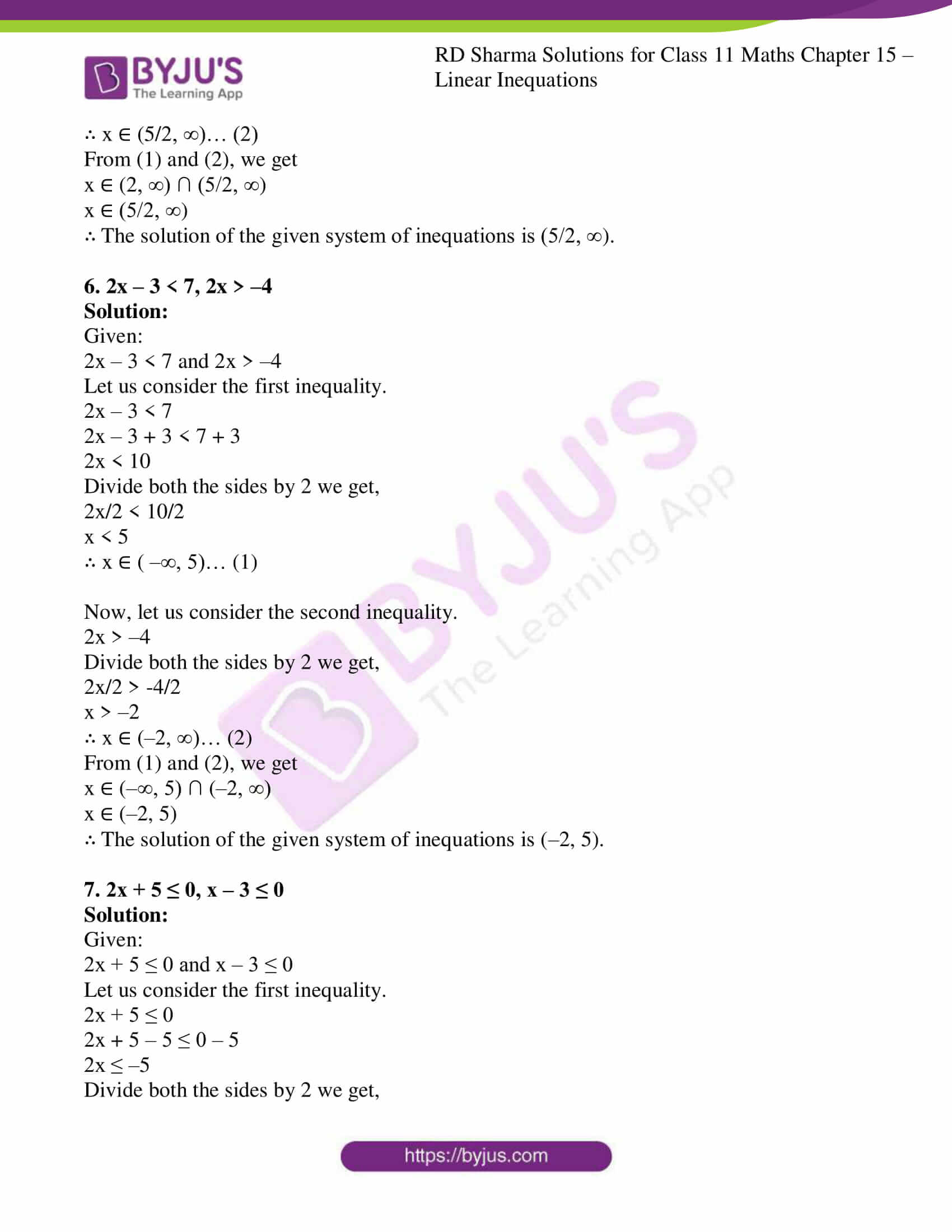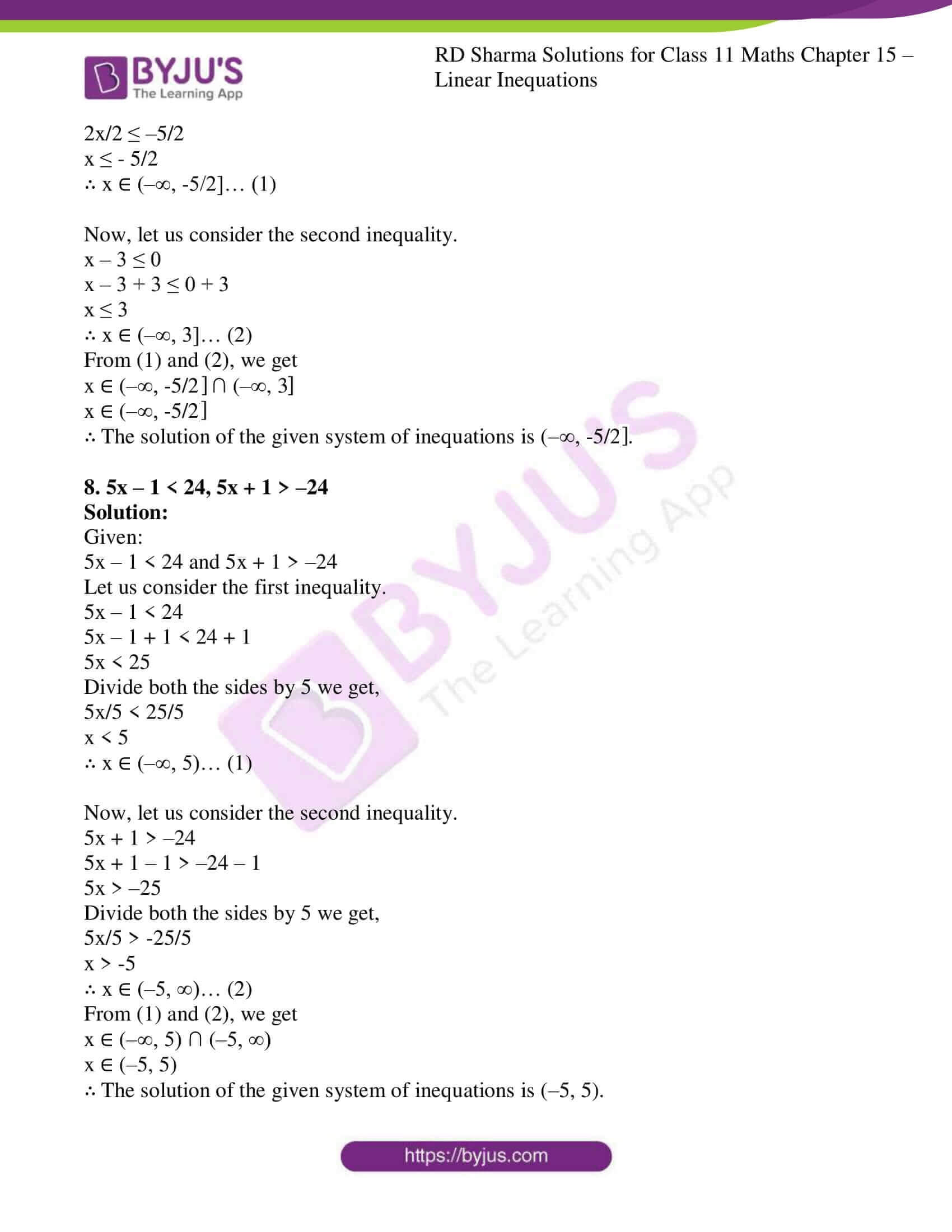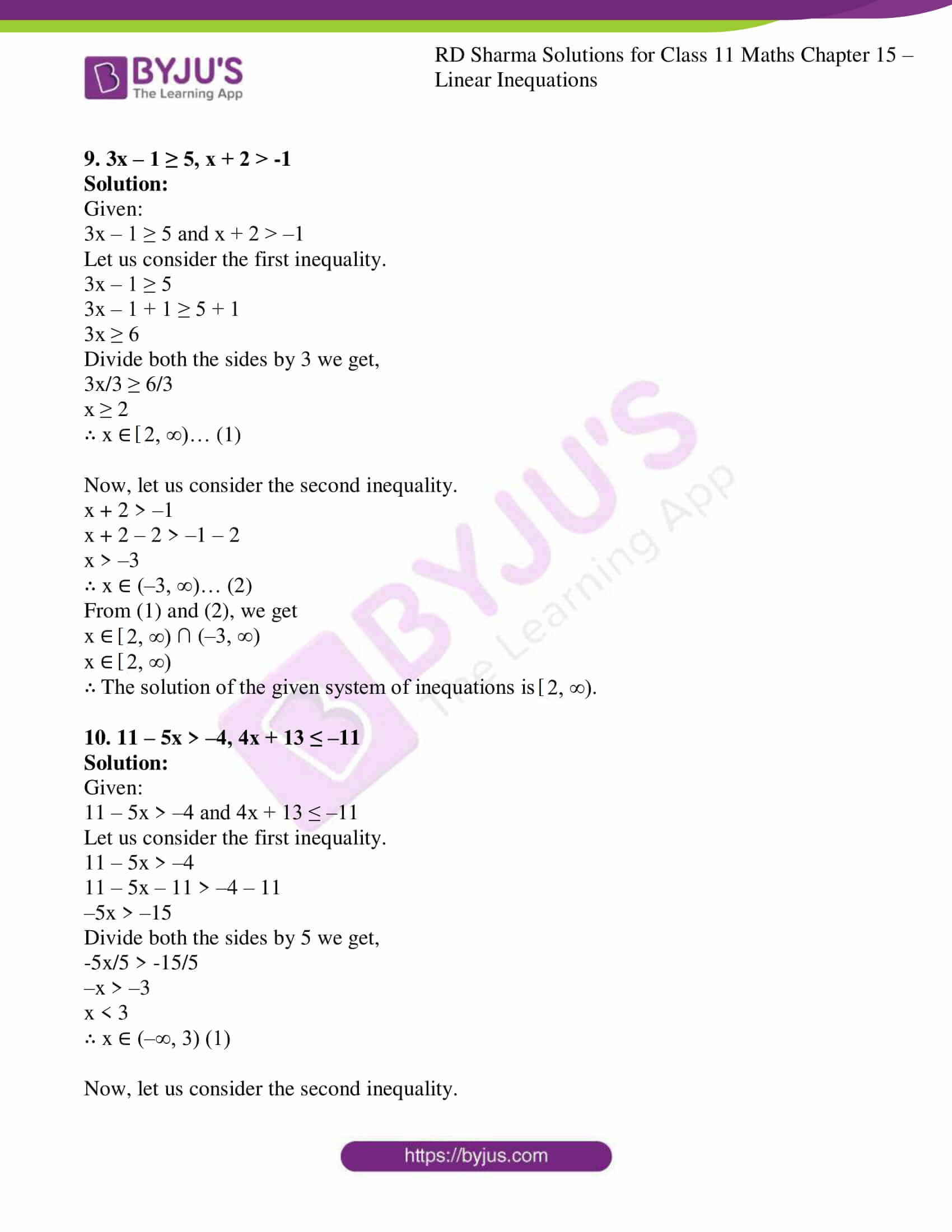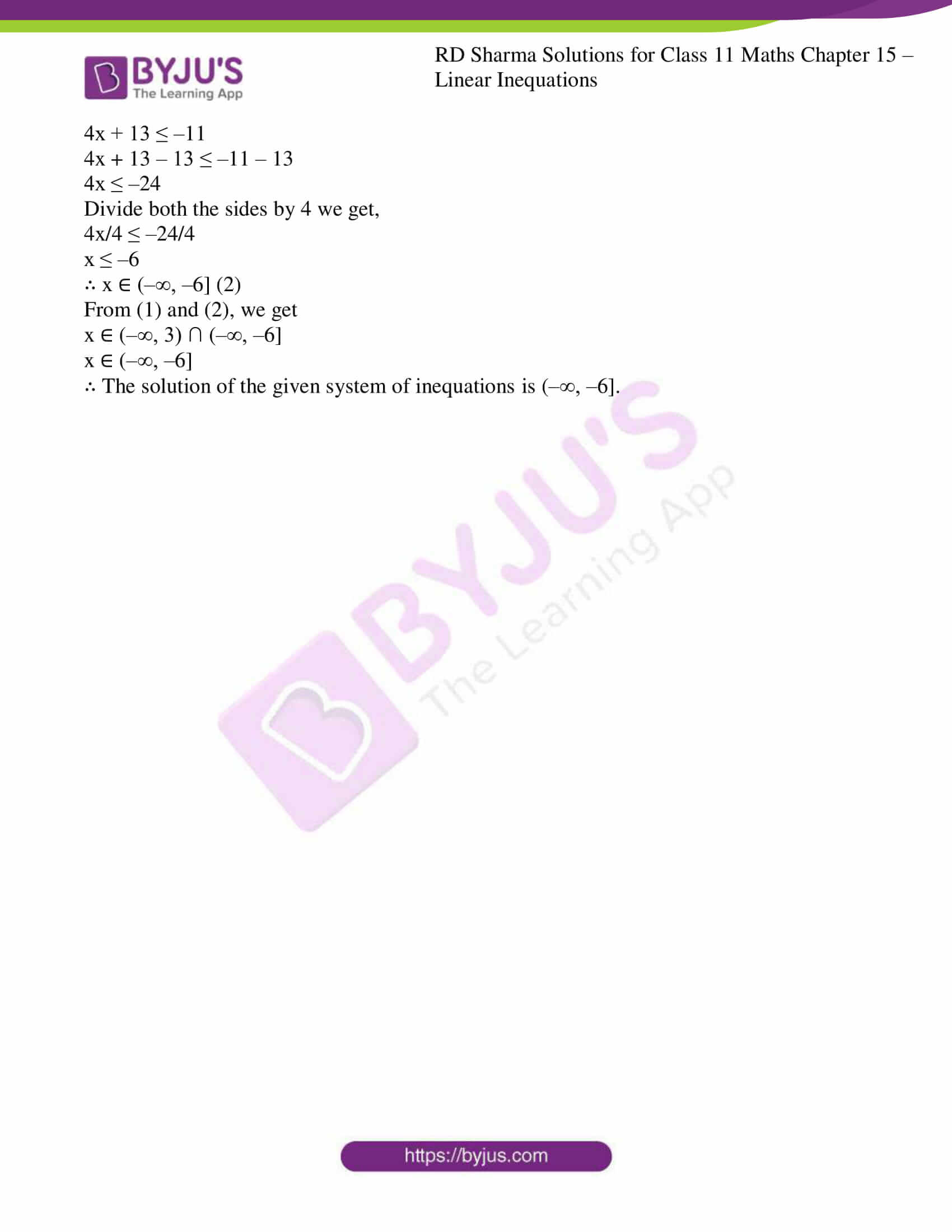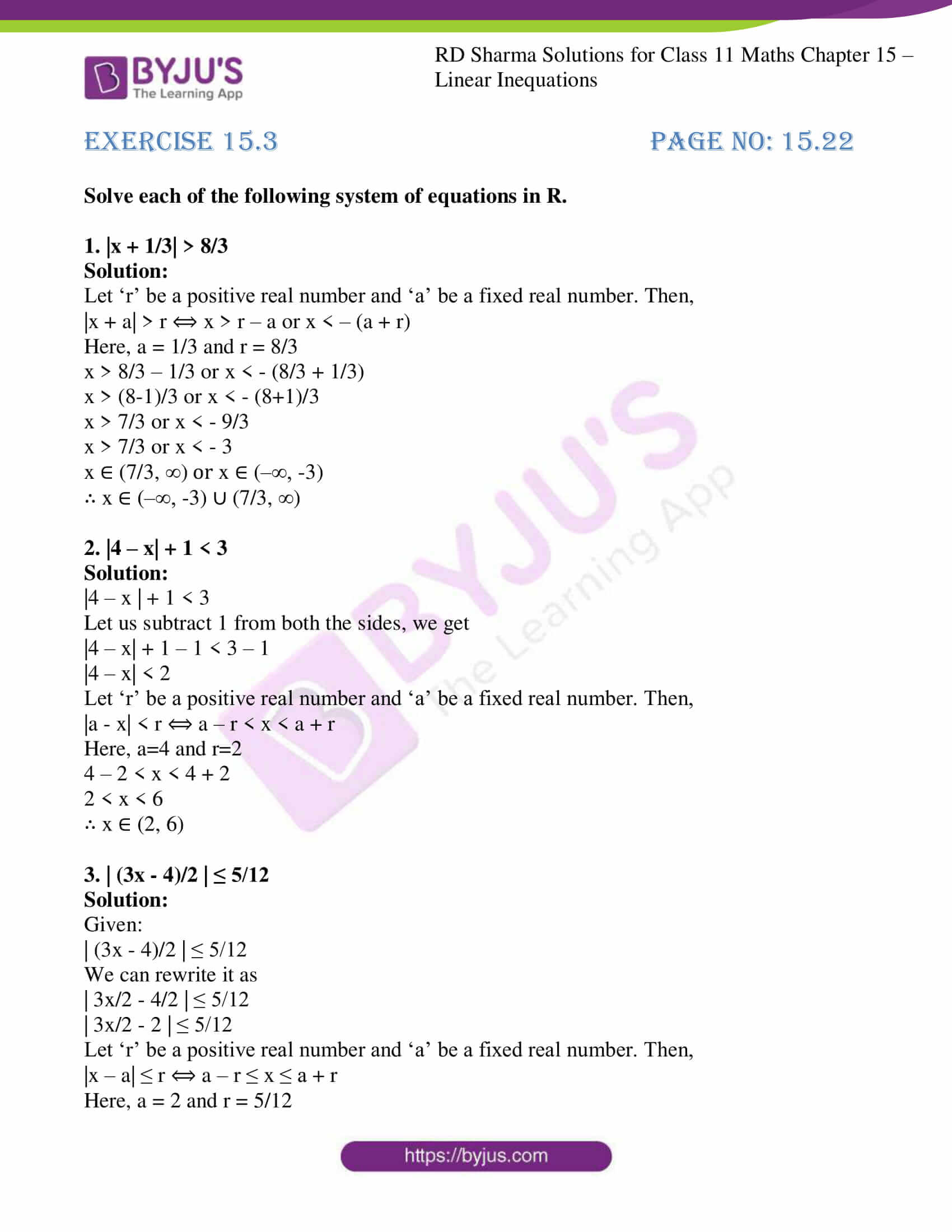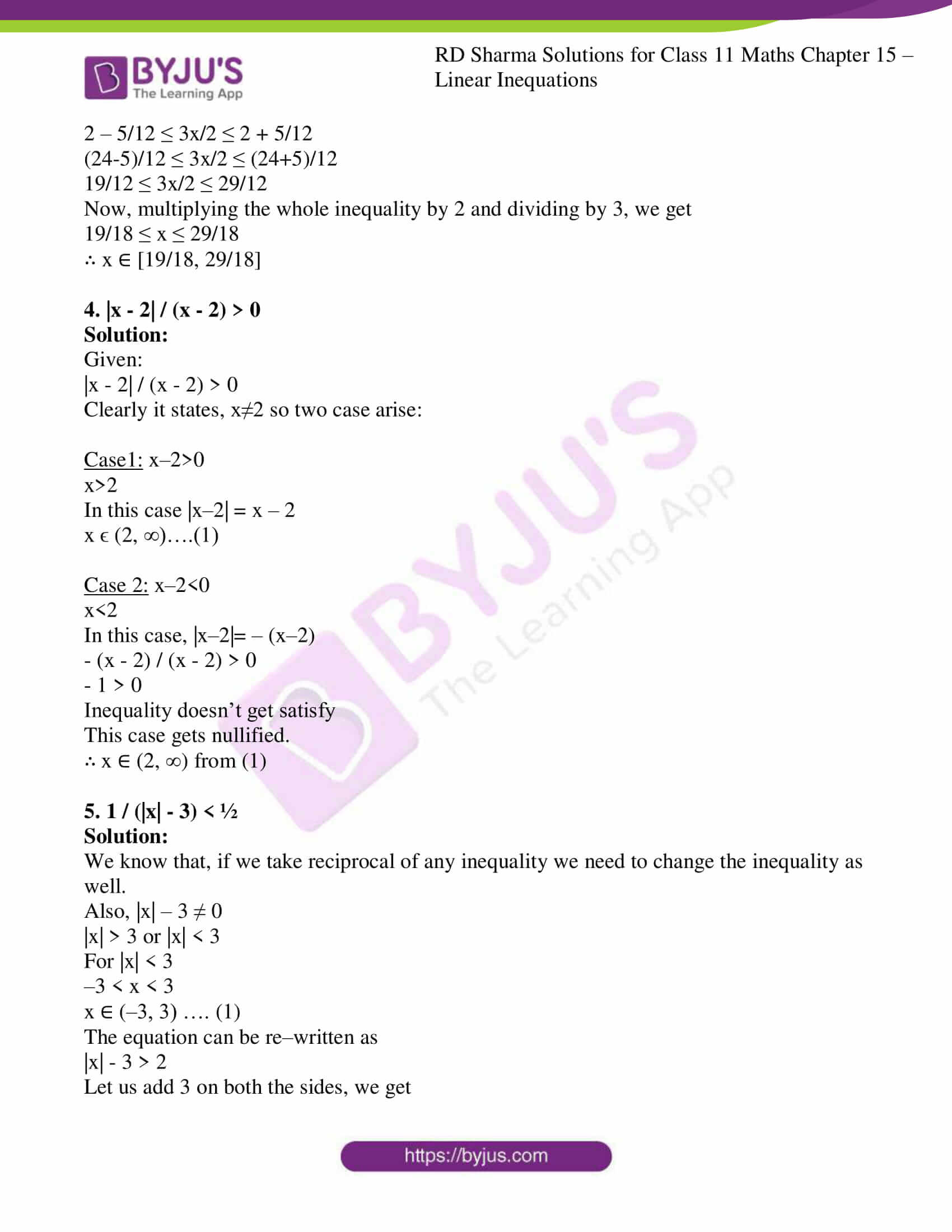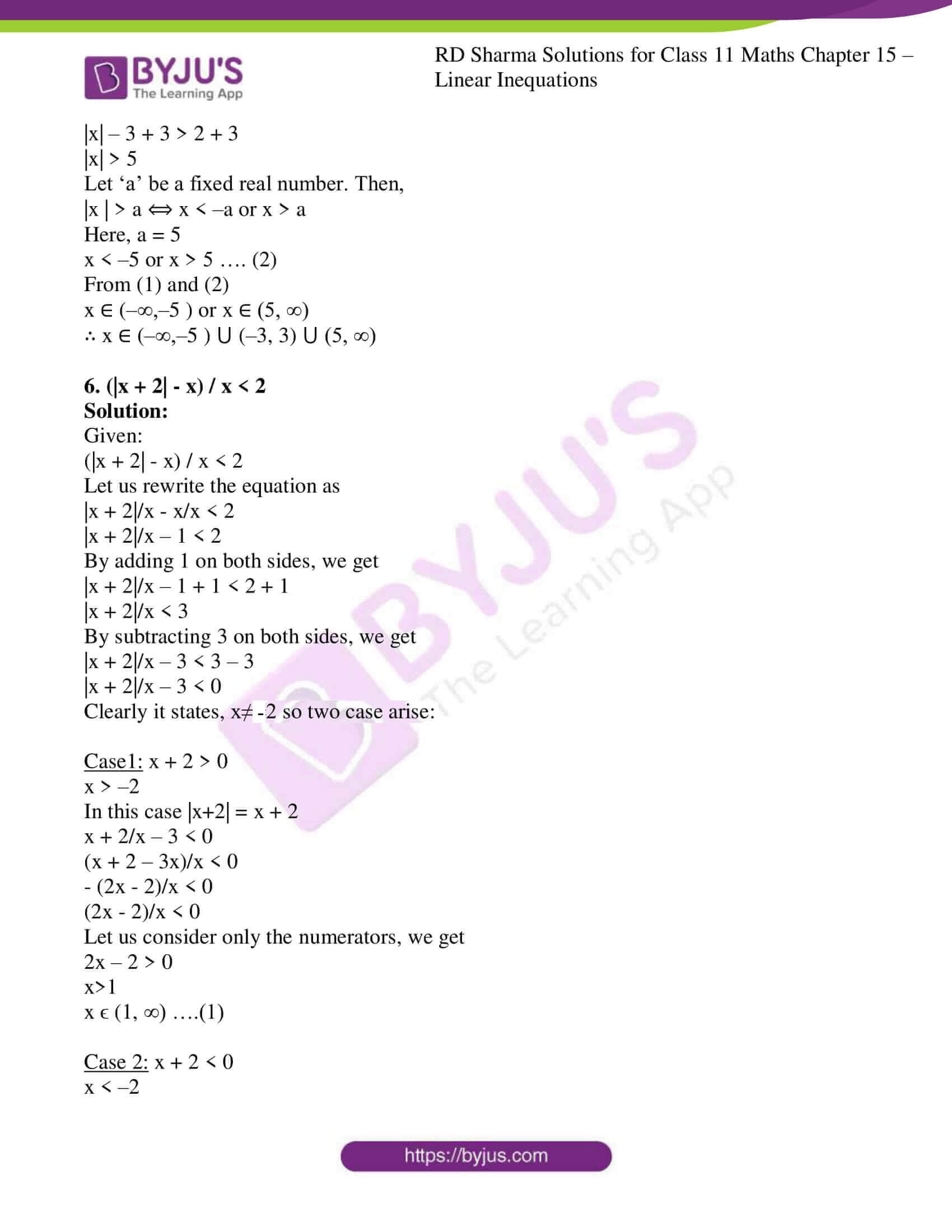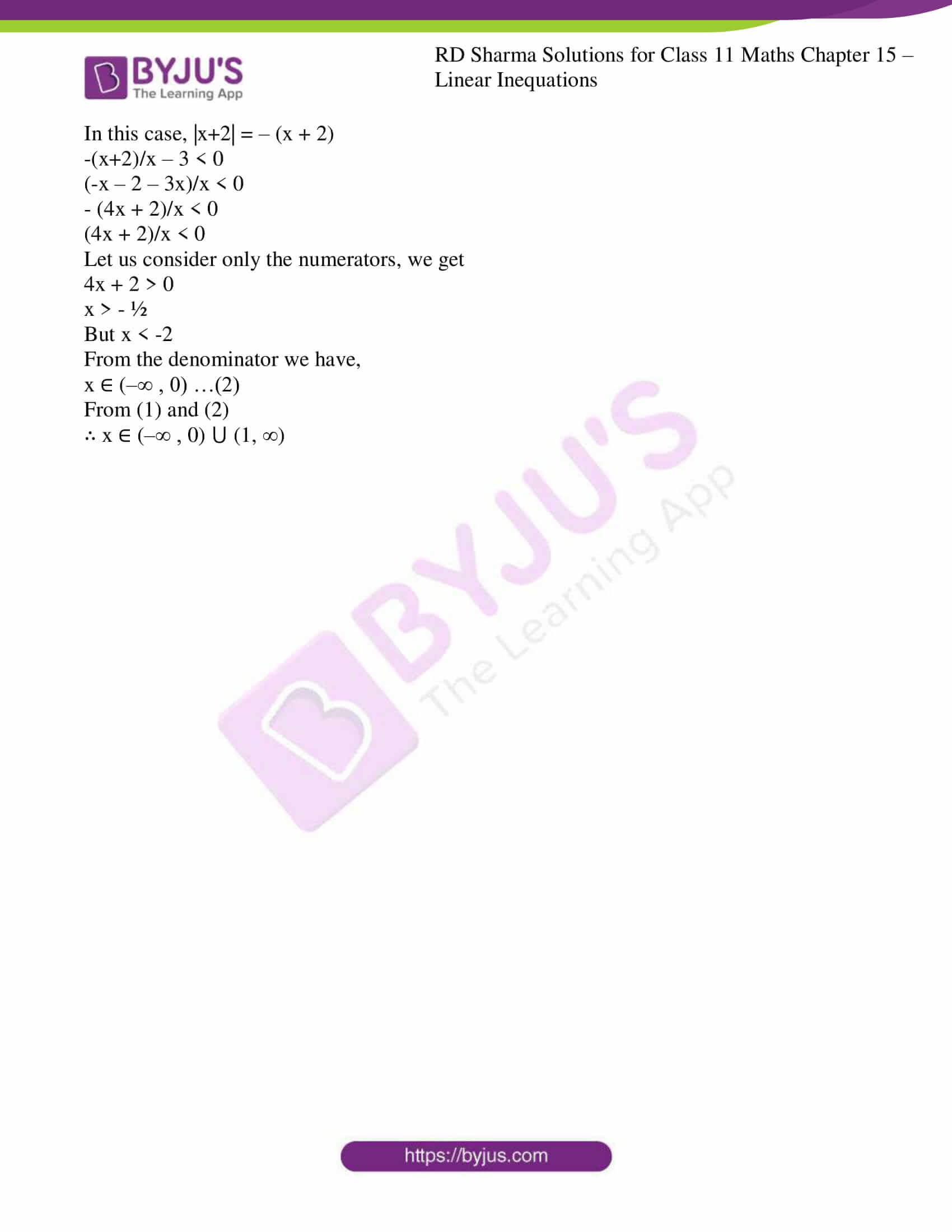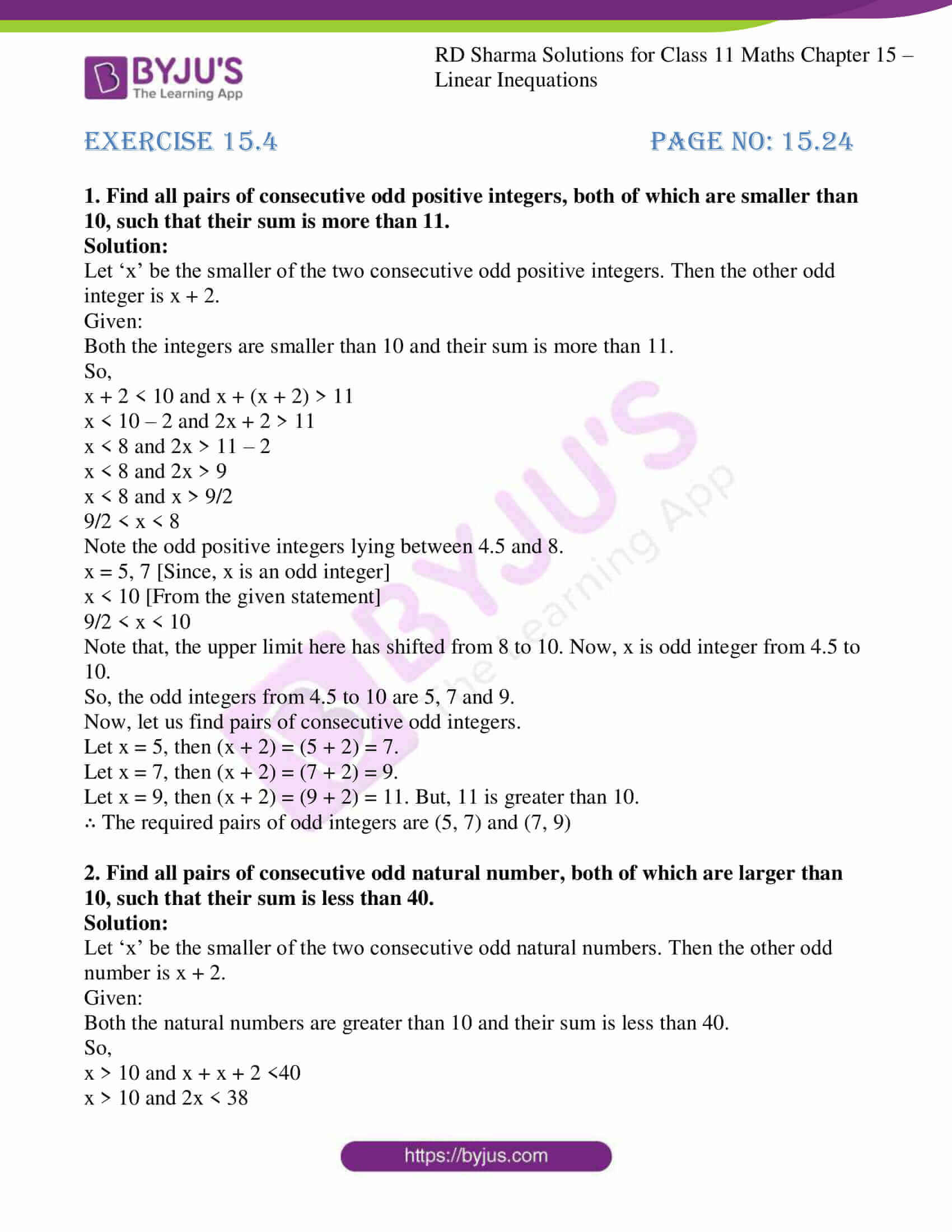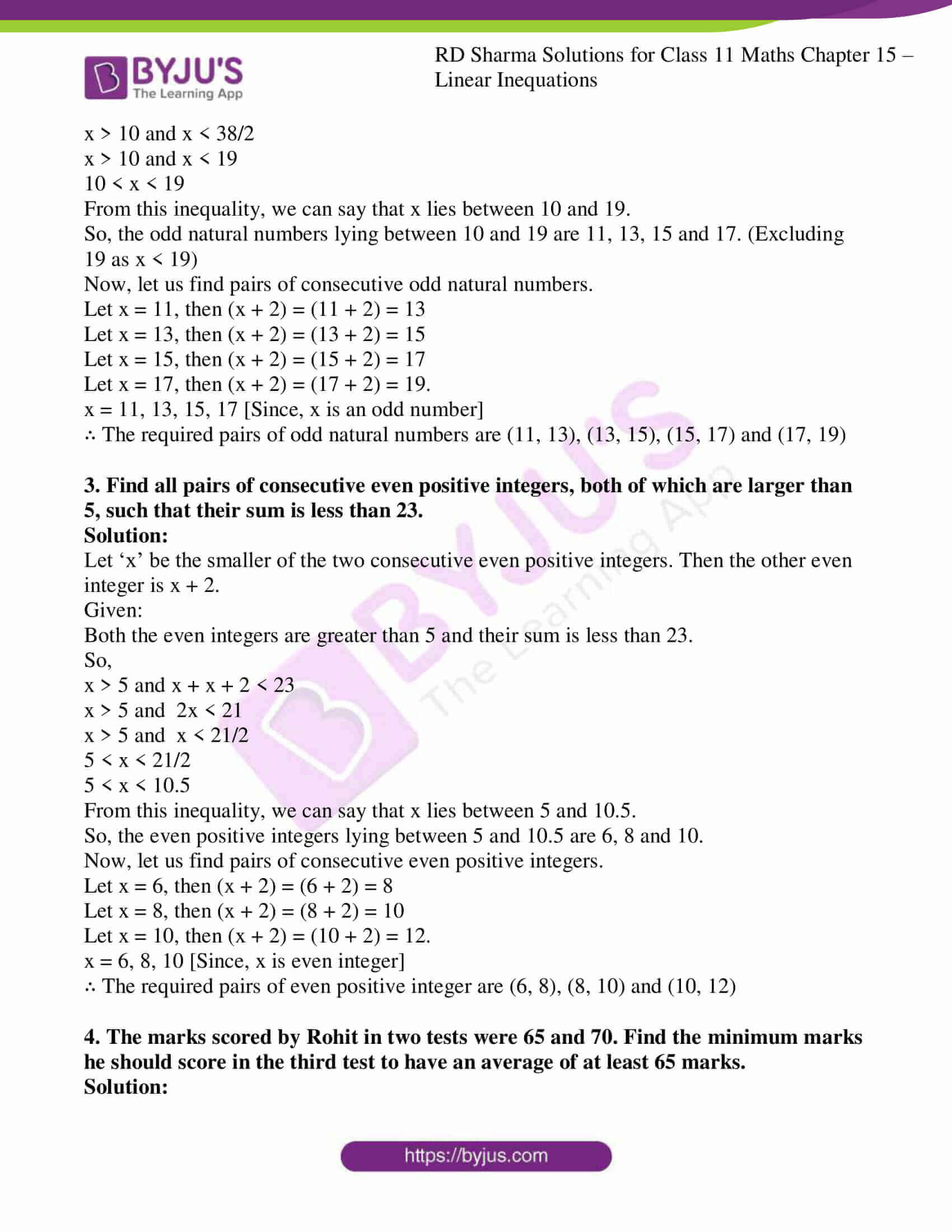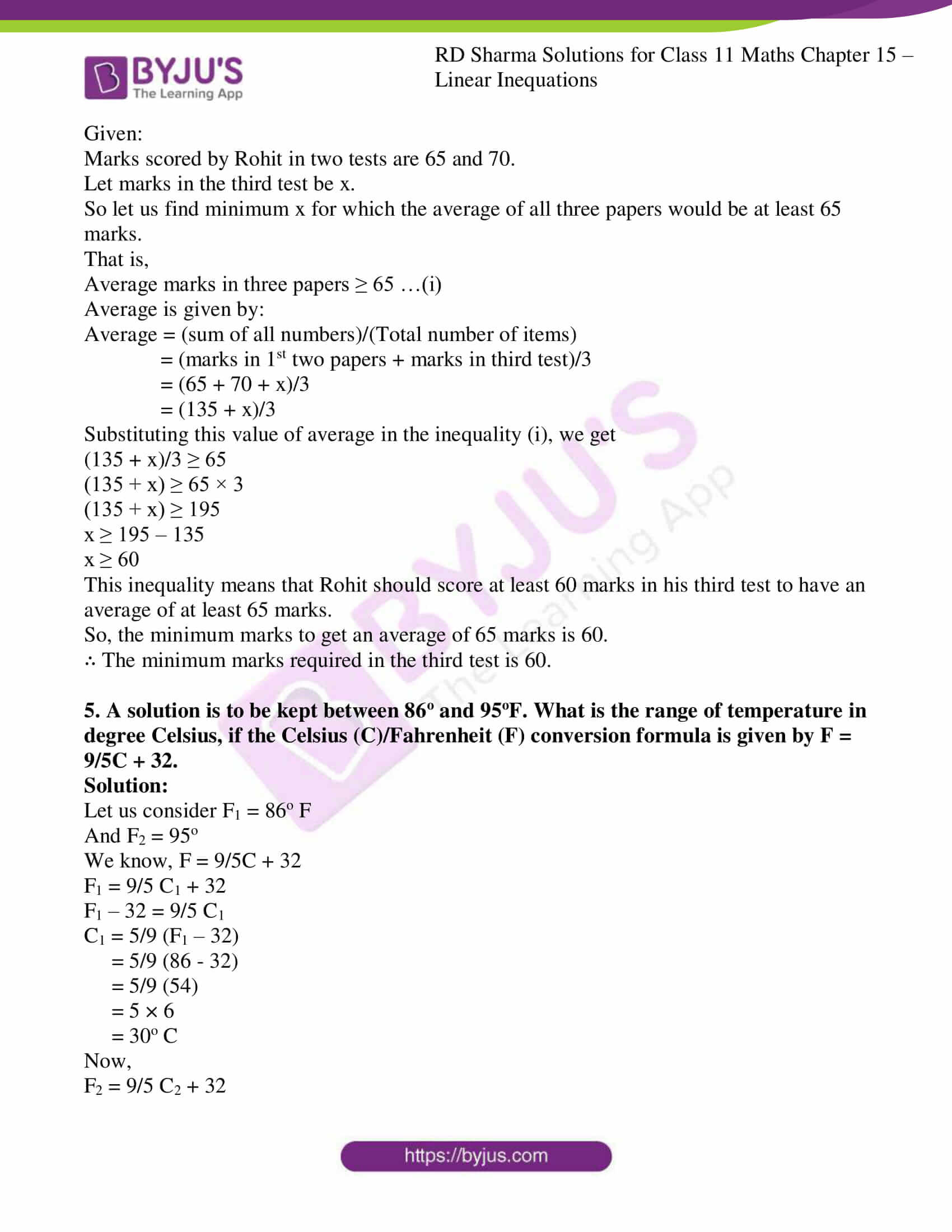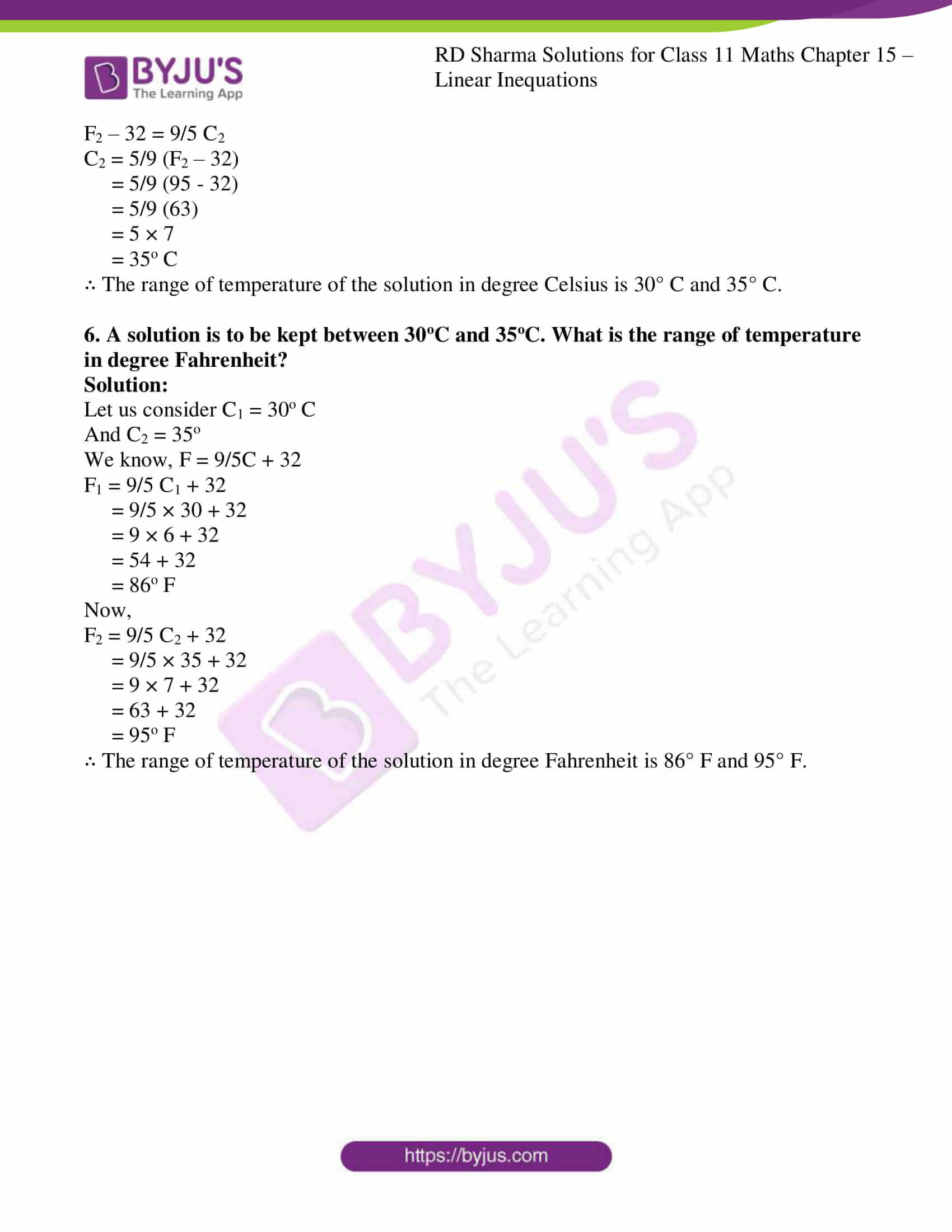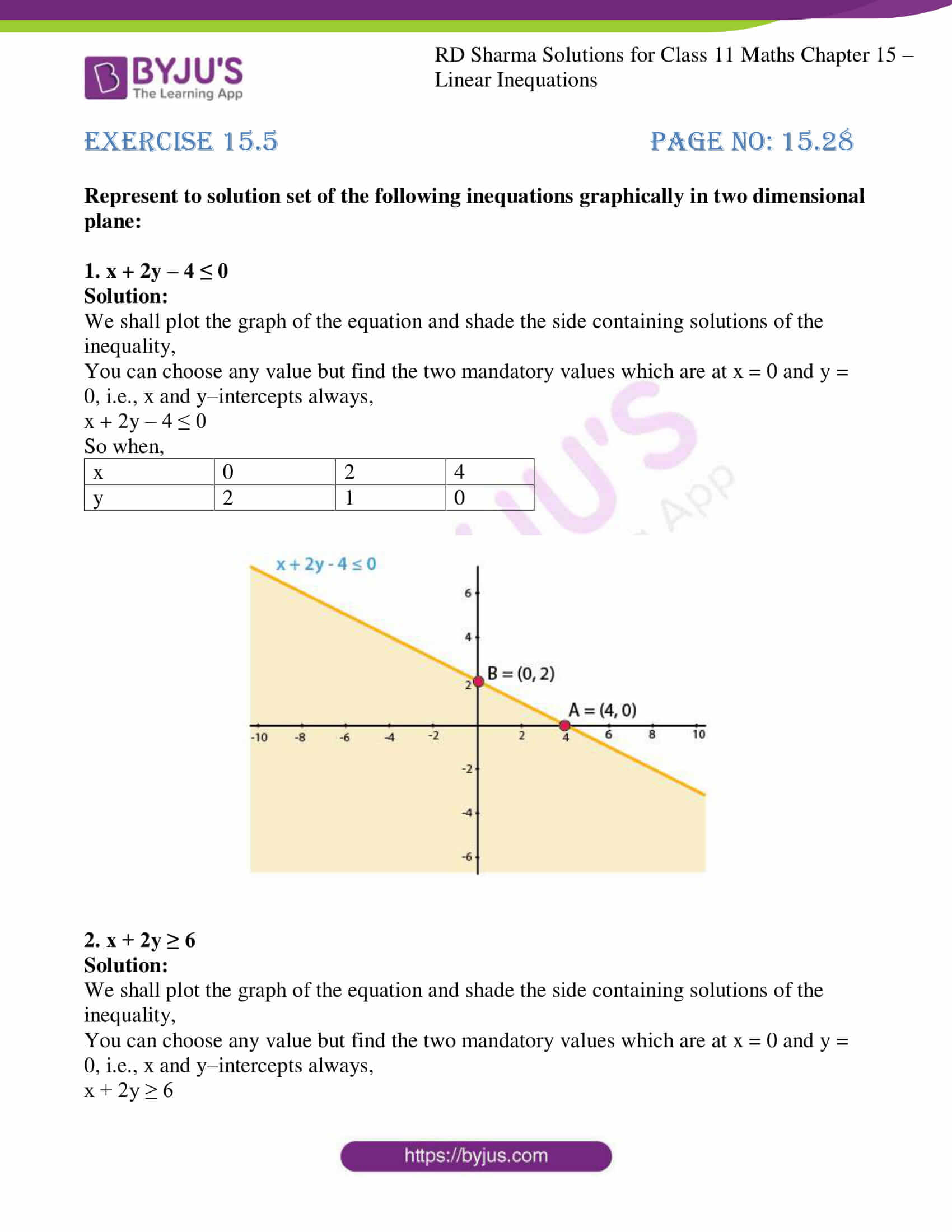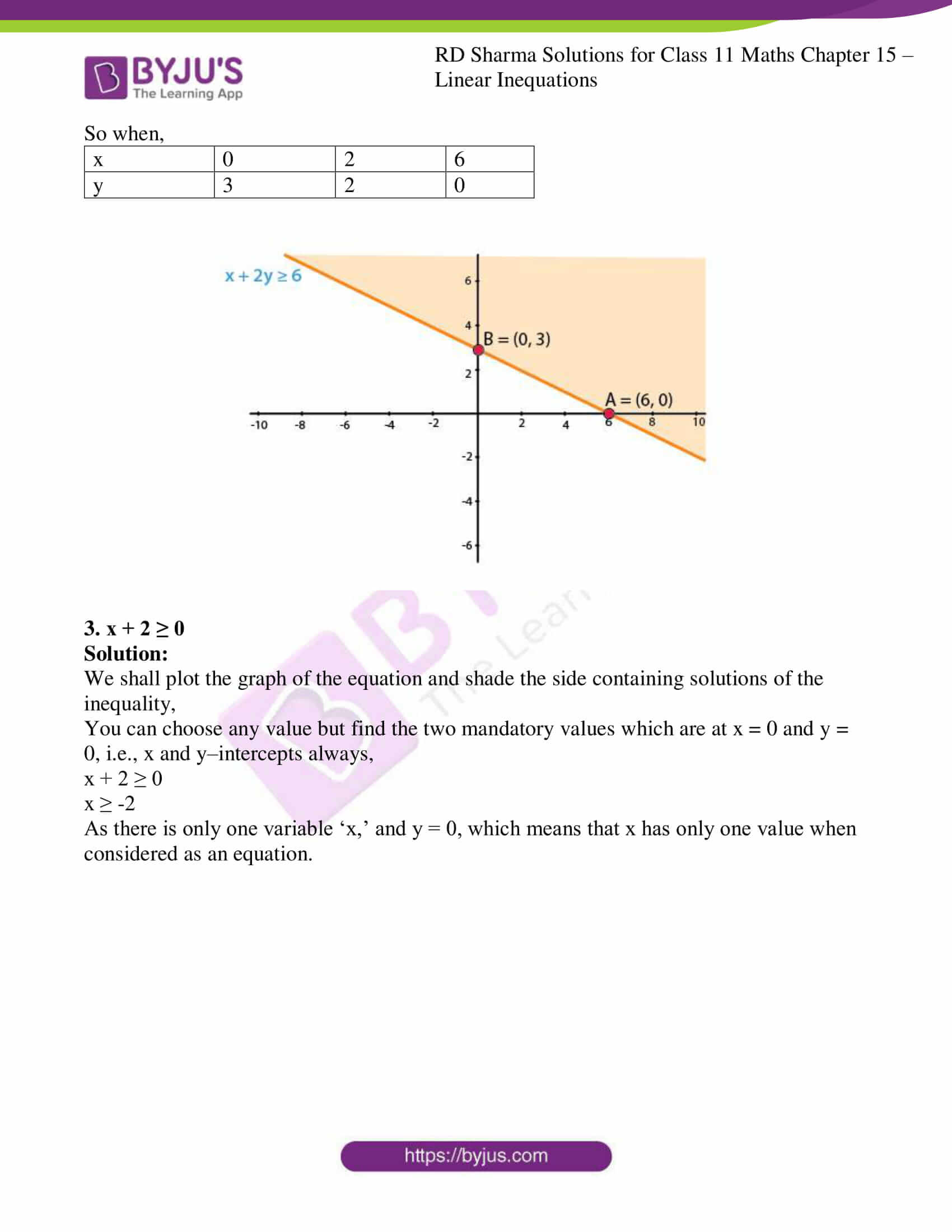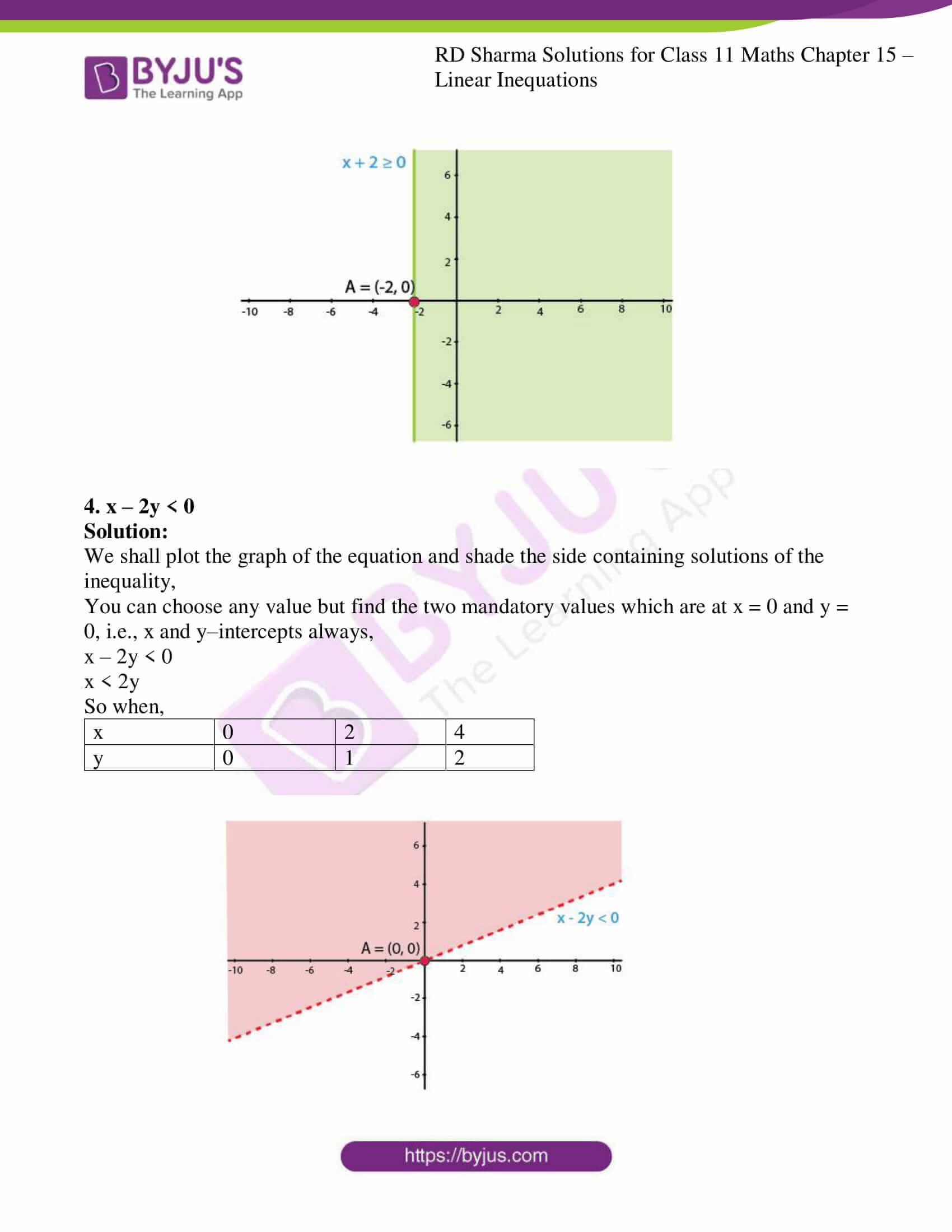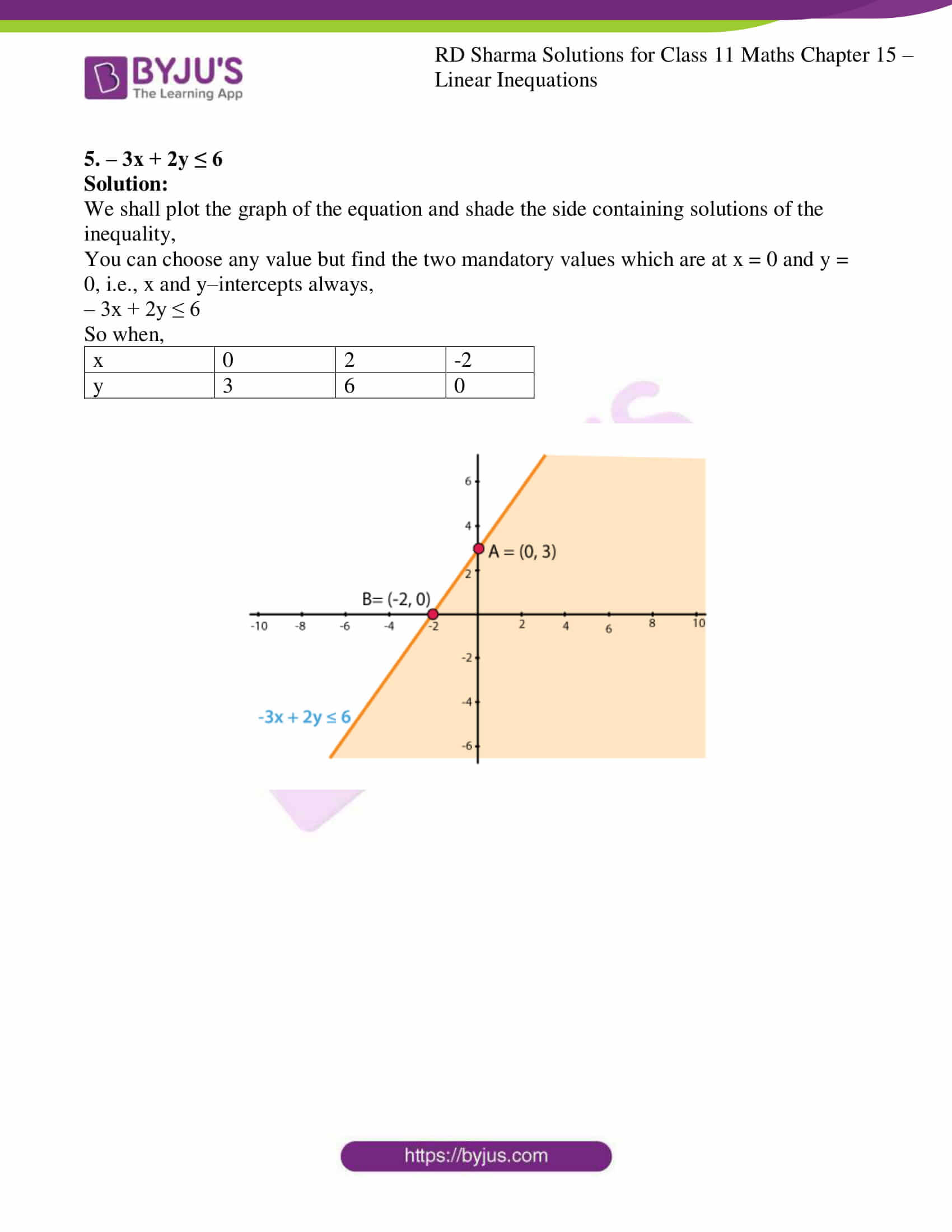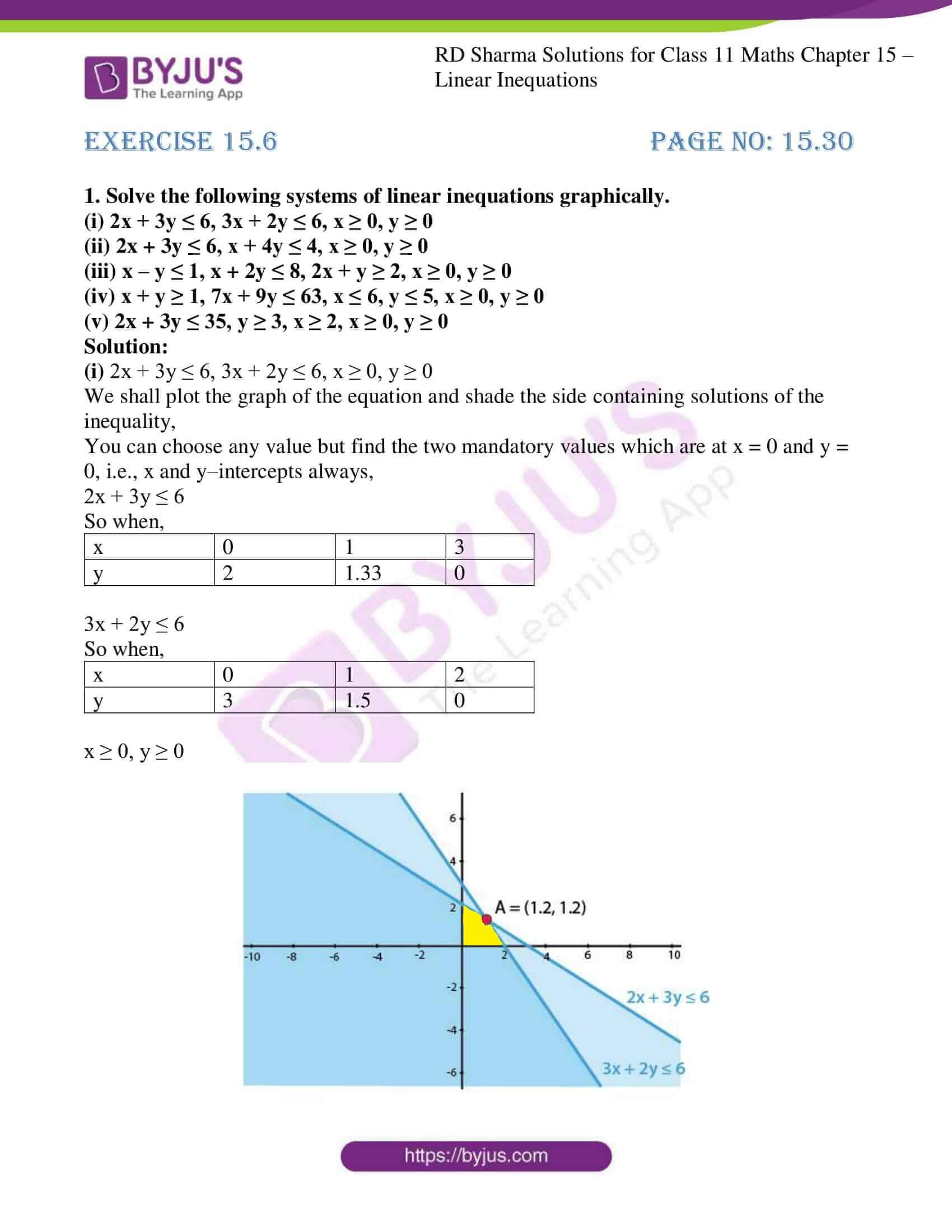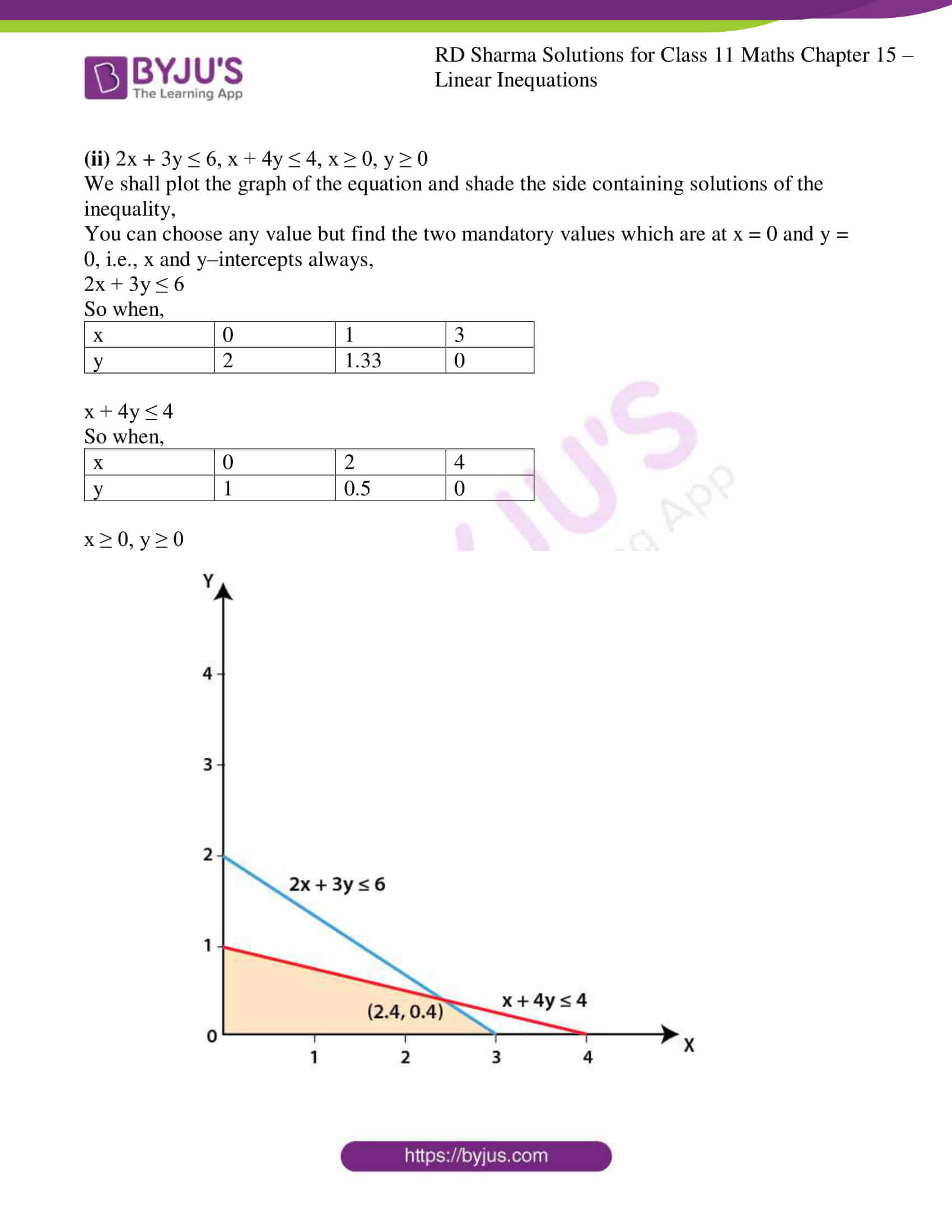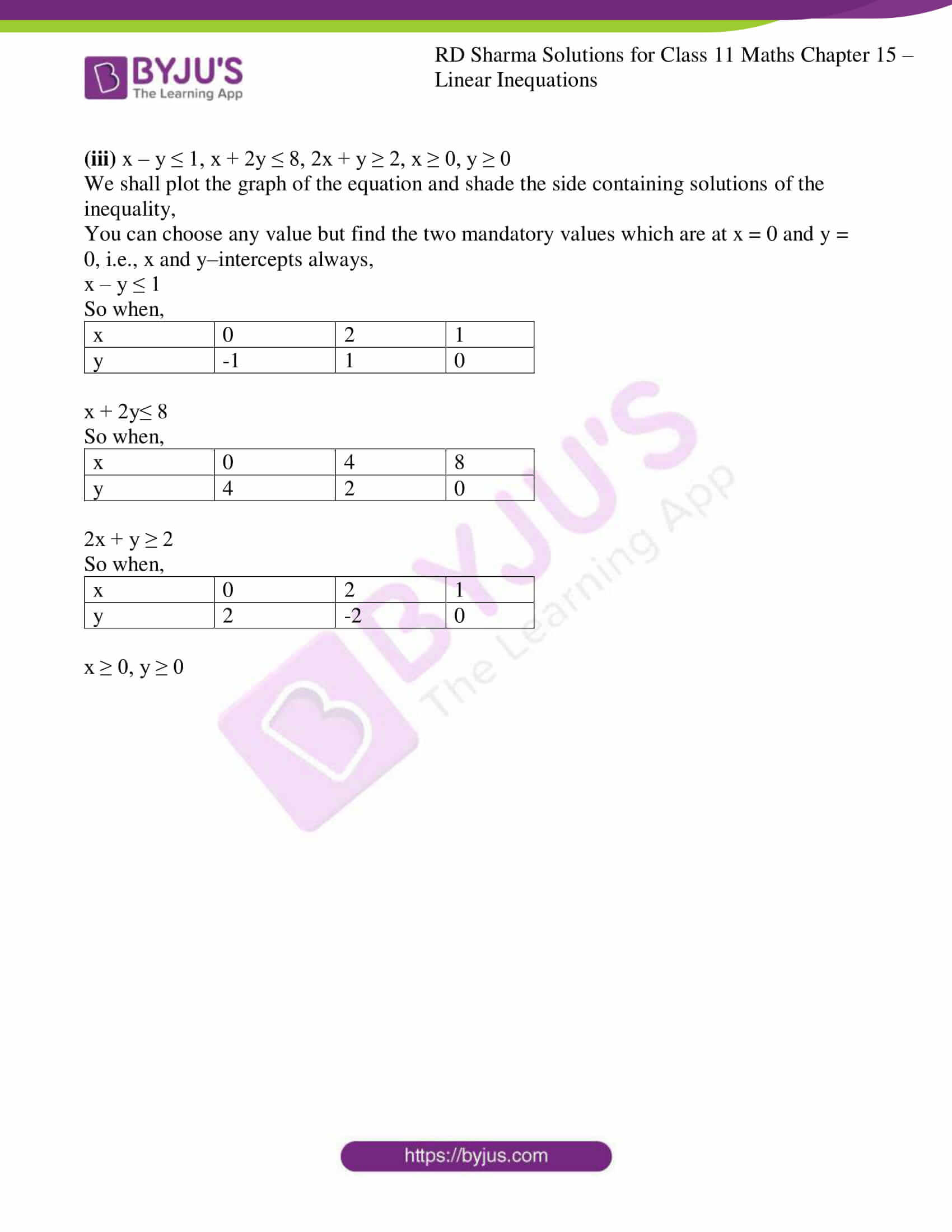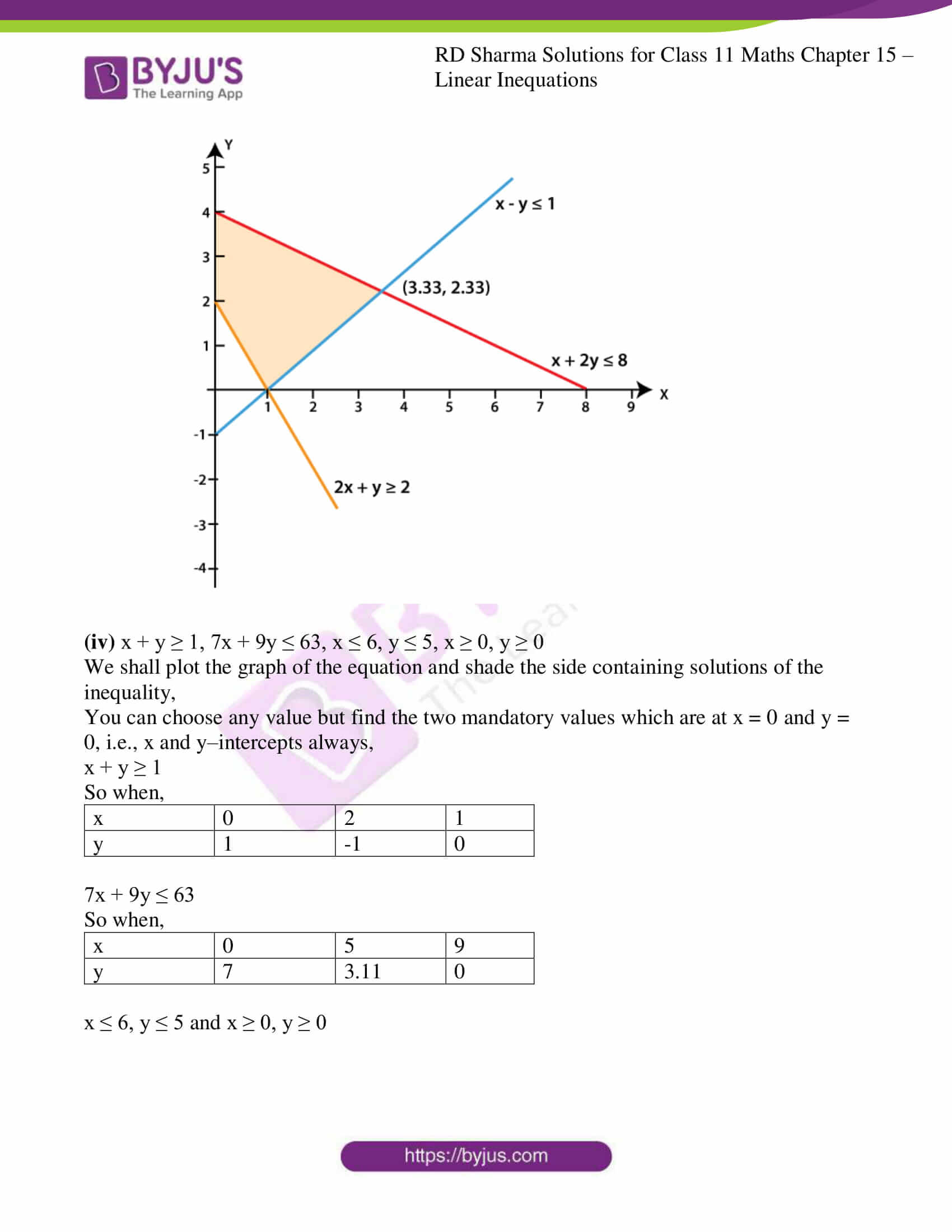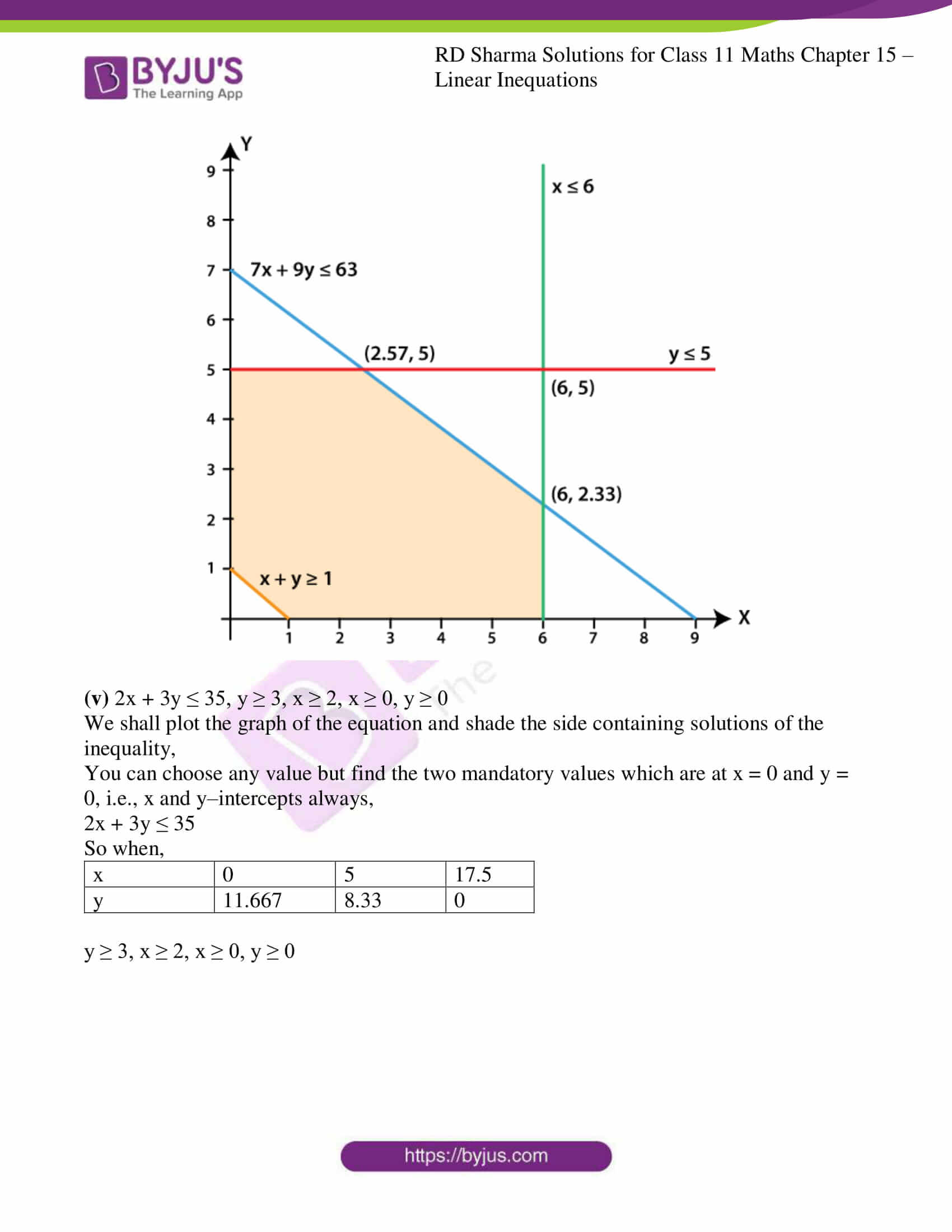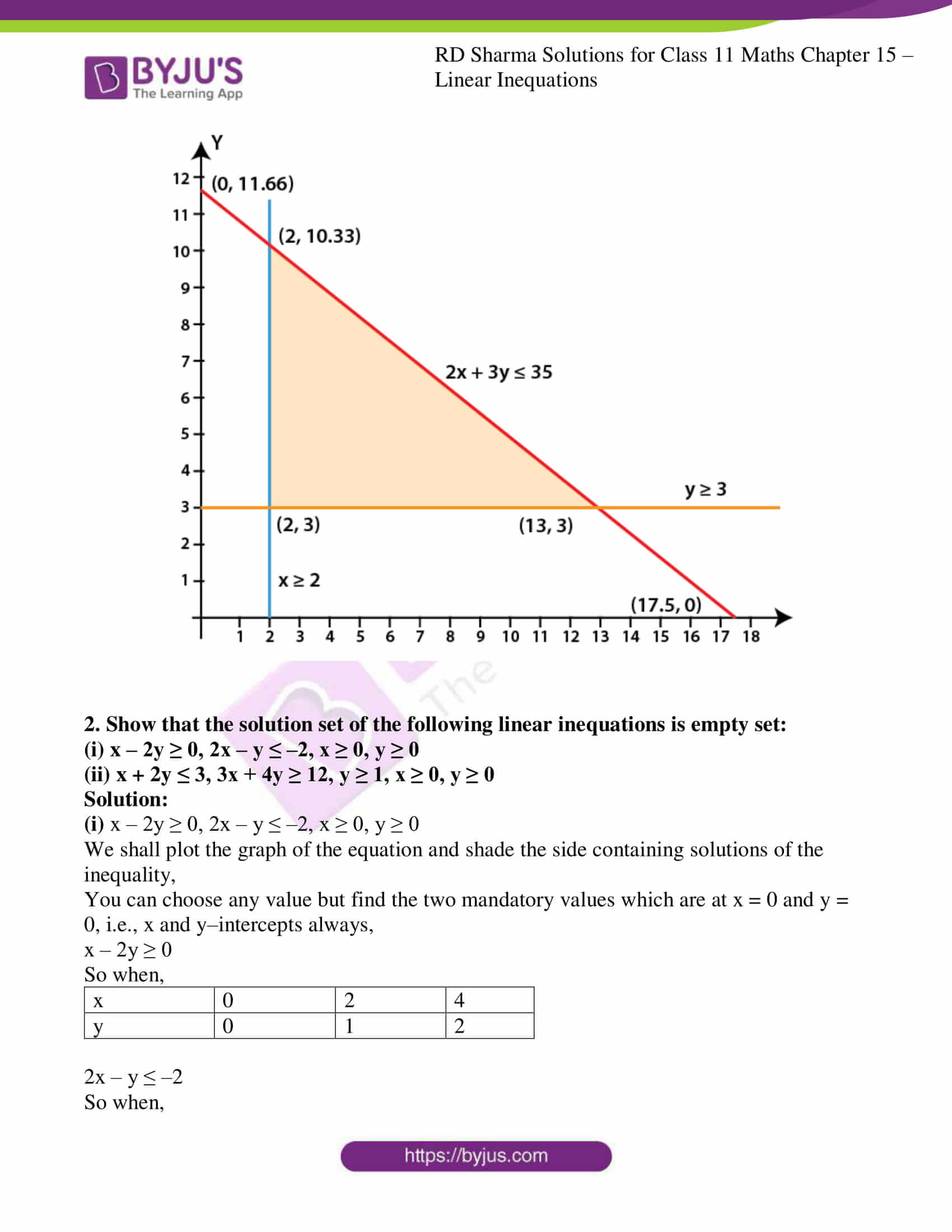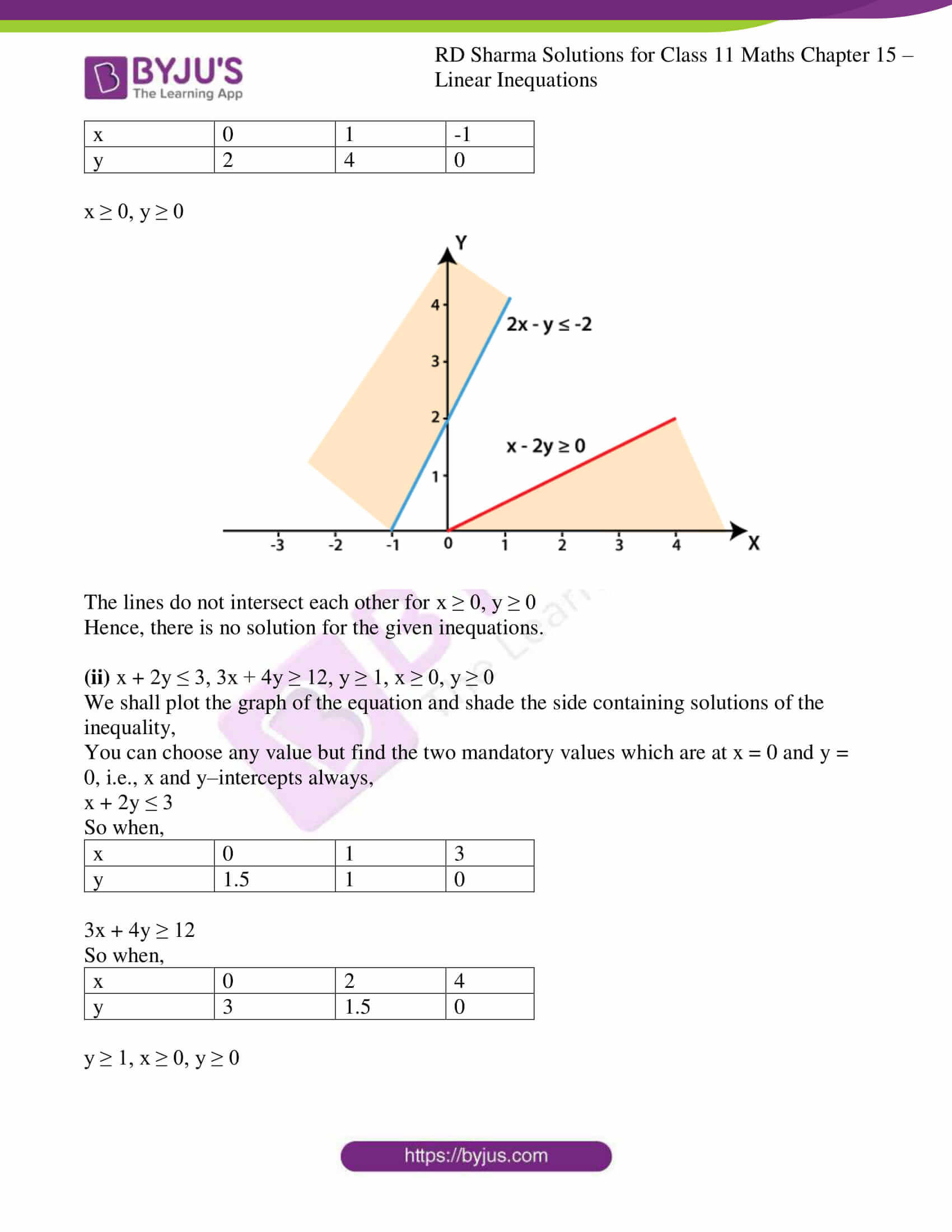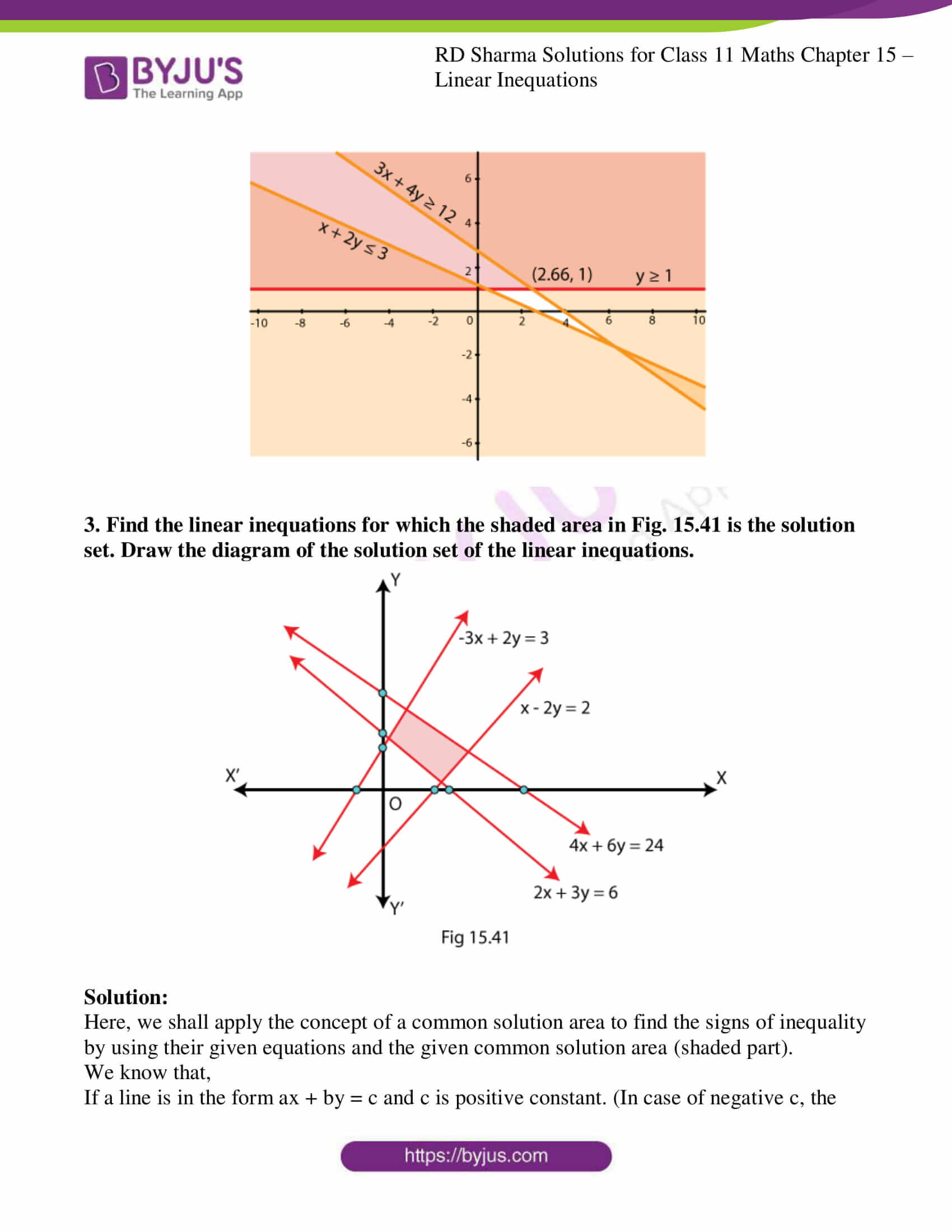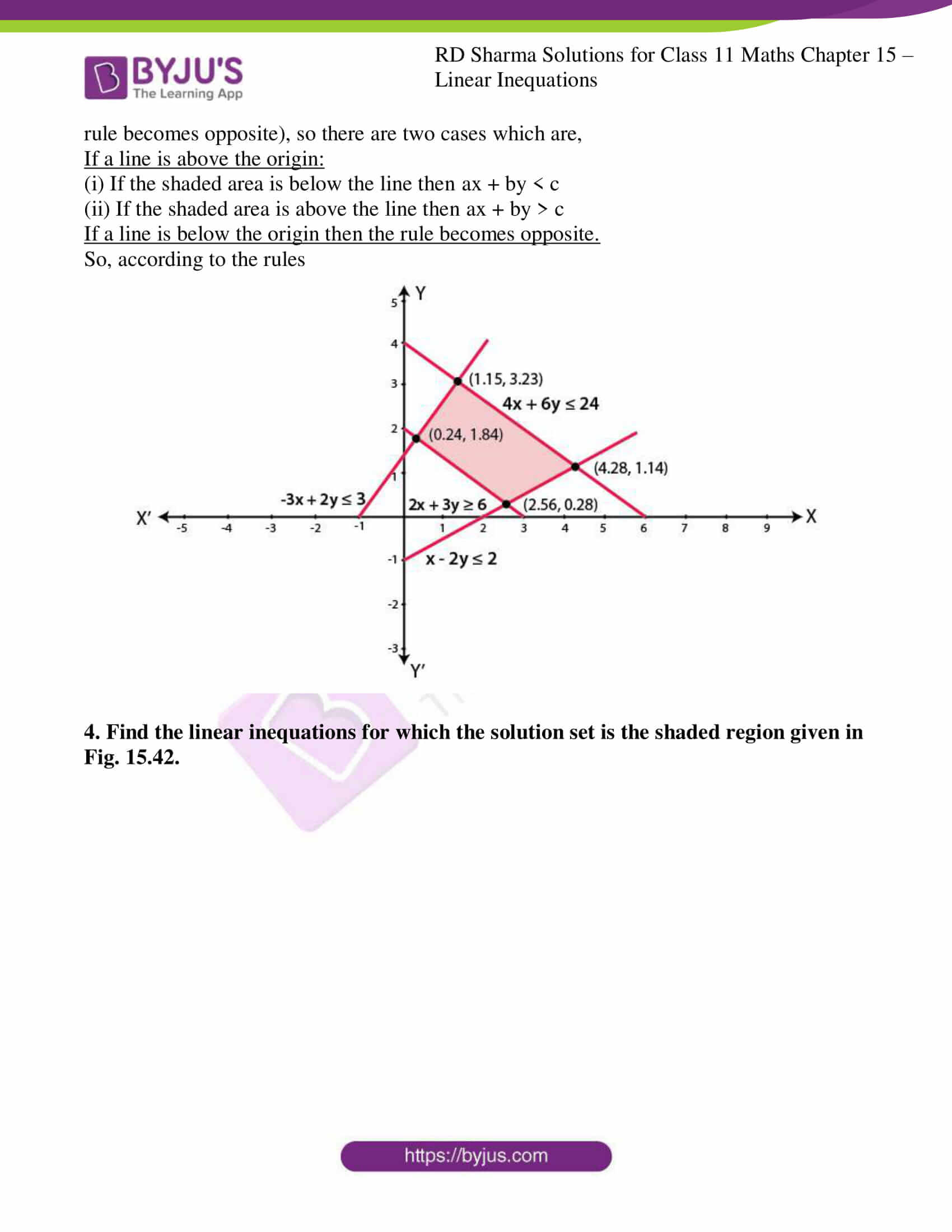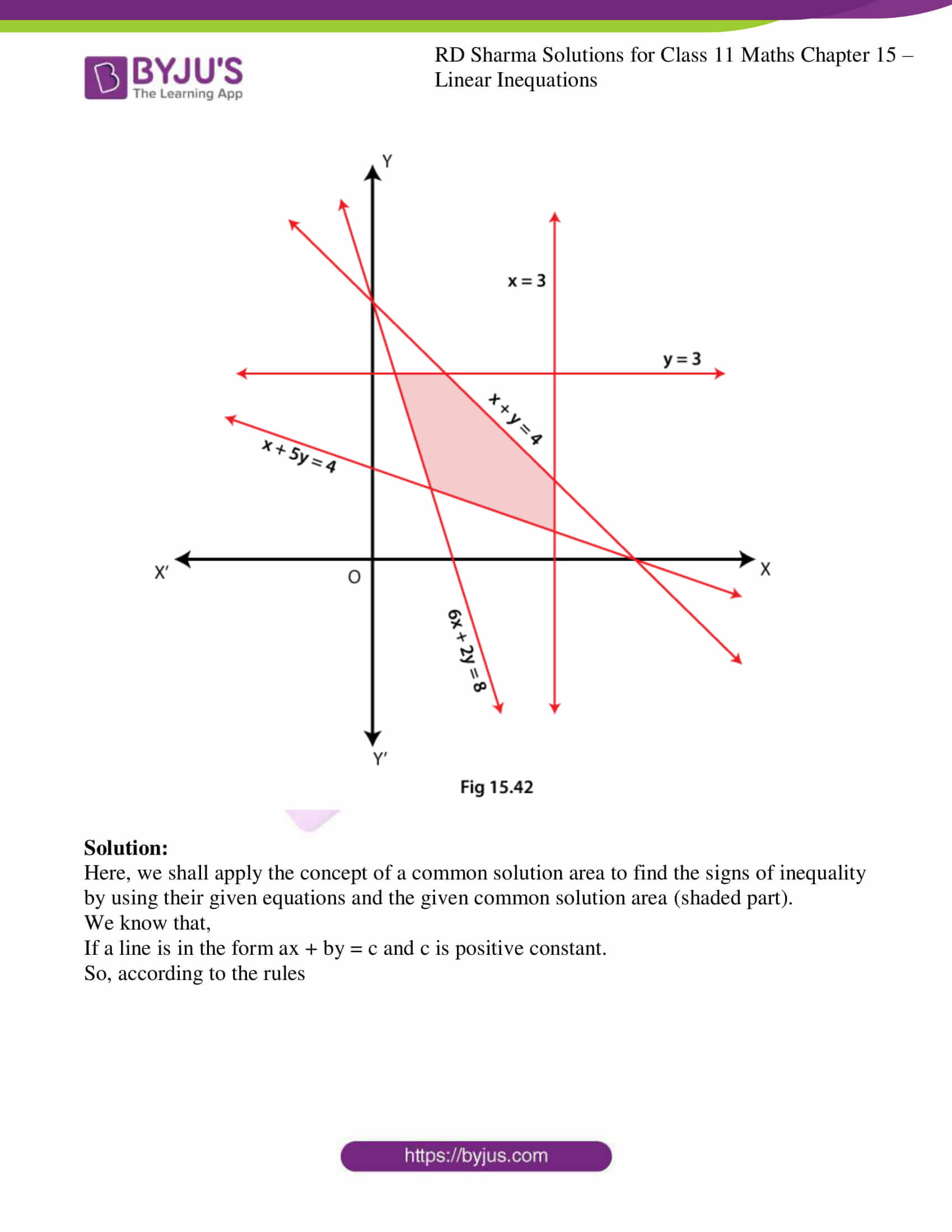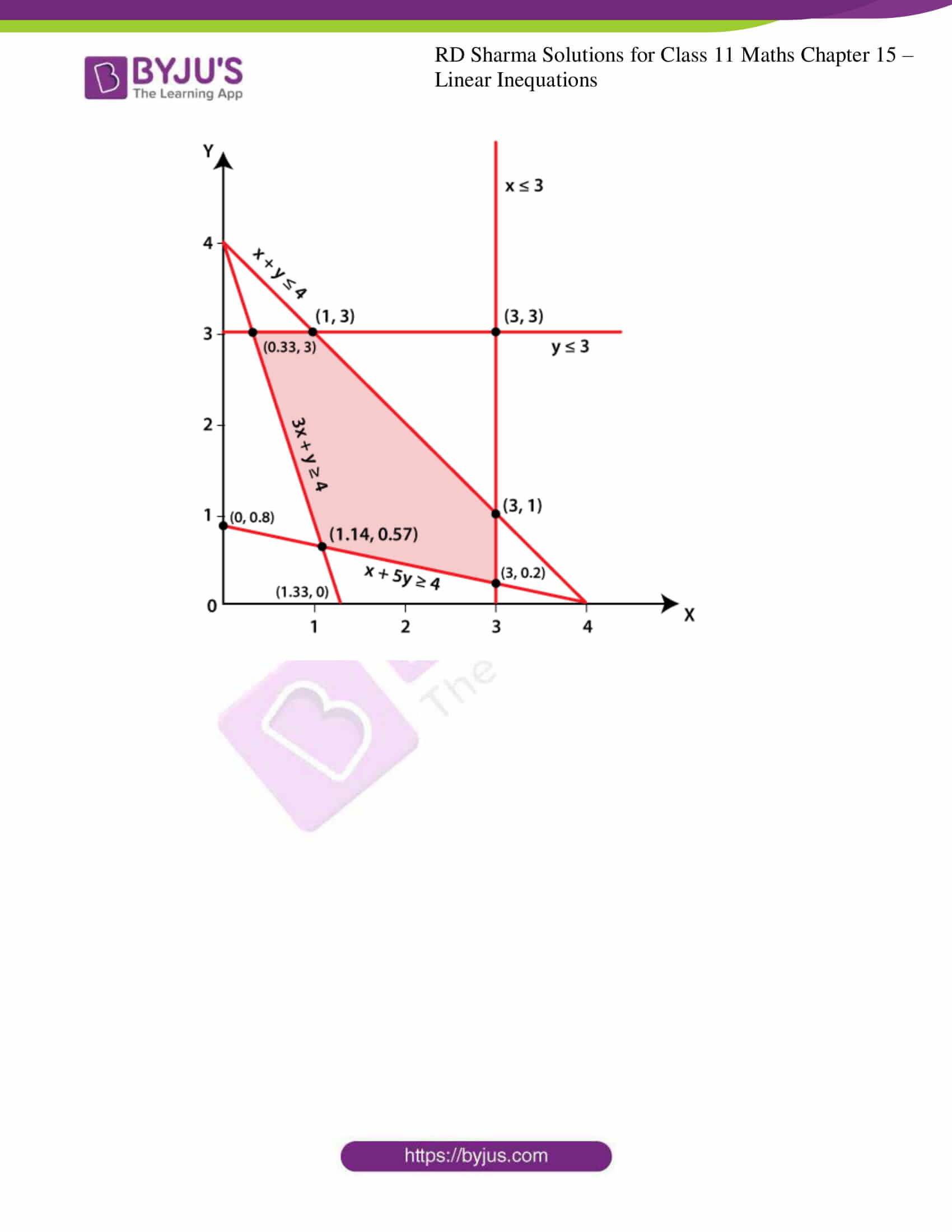### Access answers to RD Sharma Solutions for Class 11 Maths Chapter 15 – Linear Inequations

EXERCISE 15.1 PAGE NO: 15.10

Solve the following linear Inequations in R.

1. Solve: 12x < 50, when

(i) x ∈ R

(ii) x ∈ Z

(iii) x ∈ N

Solution:

Given:

12x < 50

So when we divide by 12, we get

12x/ 12 < 50/12

x < 25/6

(i) x ∈ R

When x is a real number, the solution of the given inequation is (-∞, 25/6).

(ii) x ∈ Z

When, 4 < 25/6 < 5

So when, when x is an integer, the maximum possible value of x is 4.

The solution of the given inequation is {…, –2, –1, 0, 1, 2, 3, 4}.

(iii) x ∈ N

When, 4 < 25/6 < 5

So when, when x is a natural number, the maximum possible value of x is 4. We know that the natural numbers start from 1, the solution of the given inequation is {1, 2, 3, 4}.

2. Solve: -4x > 30, when

(i) x ∈ R

(ii) x ∈ Z

(iii) x ∈ N

Solution:

Given:

-4x > 30

So when we divide by 4, we get

-4x/4 > 30/4

-x > 15/2

x < – 15/2

(i) x ∈ R

When x is a real number, the solution of the given inequation is (-∞, -15/2).

(ii) x ∈ Z

When, -8 < -15/2 < -7

So when, when x is an integer, the maximum possible value of x is -8.

The solution of the given inequation is {…, –11, –10, -9, -8}.

(iii) x ∈ N

As natural numbers start from 1 and can never be negative, when x is a natural number, the solution of the given inequation is ∅.

3. Solve: 4x-2 < 8, when

(i) x ∈ R

(ii) x ∈ Z

(iii) x ∈ N

Solution:

Given:

4x – 2 < 8

4x – 2 + 2 < 8 + 2

4x < 10

So divide by 4 on both sides we get,

4x/4 < 10/4

x < 5/2

(i) x ∈ R

When x is a real number, the solution of the given inequation is (-∞, 5/2).

(ii) x ∈ Z

When, 2 < 5/2 < 3

So when, when x is an integer, the maximum possible value of x is 2.

The solution of the given inequation is {…, –2, –1, 0, 1, 2}.

(iii) x ∈ N

When, 2 < 5/2 < 3

So when, when x is a natural number, the maximum possible value of x is 2. We know that the natural numbers start from 1, the solution of the given inequation is {1, 2}.

4. 3x – 7 > x + 1

Solution:

Given:

3x – 7 > x + 1

3x – 7 + 7 > x + 1 + 7

3x > x + 8

3x – x > x + 8 – x

2x > 8

Divide both sides by 2, we get

2x/2 > 8/2

x > 4

∴ The solution of the given inequation is (4, ∞).

5. x + 5 > 4x – 10

Solution:

Given: x + 5 > 4x – 10

x + 5 – 5 > 4x – 10 – 5

x > 4x – 15

4x – 15 < x

4x – 15 – x < x – x

3x – 15 < 0

3x – 15 + 15 < 0 + 15

3x < 15

Divide both sides by 3, we get

3x/3 < 15/3

x < 5

∴ The solution of the given inequation is (-∞, 5).

6. 3x + 9 ≥ –x + 19

Solution:

Given: 3x + 9 ≥ –x + 19

3x + 9 – 9 ≥ –x + 19 – 9

3x ≥ –x + 10

3x + x ≥ –x + 10 + x

4x ≥ 10

Divide both sides by 4, we get

4x/4 ≥ 10/4

x ≥ 5/2

∴ The solution of the given inequation is [5/2, ∞).

7. 2 (3 – x) ≥ x/5 + 4

Solution:

Given: 2 (3 – x) ≥ x/5 + 4

6 – 2x ≥ x/5 + 4

6 – 2x ≥ (x+20)/5

5(6 – 2x) ≥ (x + 20)

30 – 10x ≥ x + 20

30 – 20 ≥ x + 10x

10 ≥11x

11x ≤ 10

Divide both sides by 11, we get

11x/11 ≤ 10/11

x ≤ 10/11

∴ The solution of the given inequation is (-∞, 10/11].

8. (3x – 2)/5 ≤ (4x – 3)/2

Solution:

Given:

(3x – 2)/5 ≤ (4x – 3)/2

Multiply both the sides by 5 we get,

(3x – 2)/5 × 5 ≤ (4x – 3)/2 × 5

(3x – 2) ≤ 5(4x – 3)/2

3x – 2 ≤ (20x – 15)/2

Multiply both the sides by 2 we get,

(3x – 2) × 2 ≤ (20x – 15)/2 × 2

6x – 4 ≤ 20x – 15

20x – 15 ≥ 6x – 4

20x – 15 + 15 ≥ 6x – 4 + 15

20x ≥ 6x + 11

20x – 6x ≥ 6x + 11 – 6x

14x ≥ 11

Divide both sides by 14, we get

14x/14 ≥ 11/14

x ≥ 11/14

∴ The solution of the given inequation is [11/14, ∞).

9. –(x – 3) + 4 < 5 – 2x

Solution:

Given: –(x – 3) + 4 < 5 – 2x

–x + 3 + 4 < 5 – 2x

–x + 7 < 5 – 2x

–x + 7 – 7 < 5 – 2x – 7

–x < –2x – 2

–x + 2x < –2x – 2 + 2x

x < –2

∴ The solution of the given inequation is (–∞, –2).

10. x/5 < (3x-2)/4 – (5x-3)/5

Solution:

Given: x/5 < (3x-2)/4 – (5x-3)/5

x/5 < [5(3x-2) – 4(5x-3)]/4(5)

x/5 < [15x – 10 – 20x + 12]/20

x/5 < [2 – 5x]/20

Multiply both the sides by 20 we get,

x/5 × 20 < [2 – 5x]/20 × 20

4x < 2 – 5x

4x + 5x < 2 – 5x + 5x

9x < 2

Divide both sides by 9, we get

9x/9 < 2/9

x < 2/9

∴ The solution of the given inequation is (-∞, 2/9).

11. [2(x-1)]/5 ≤ [3(2+x)]/7

Solution:

Given:

[2(x-1)]/5 ≤ [3(2+x)]/7

(2x – 2)/5 ≤ (6 + 3x)/7

Multiply both the sides by 5 we get,

(2x – 2)/5 × 5 ≤ (6 + 3x)/7 × 5

2x – 2 ≤ 5(6 + 3x)/7

7 (2x – 2) ≤ 5 (6 + 3x)

14x – 14 ≤ 30 + 15x

14x – 14 + 14 ≤ 30 + 15x + 14

14x ≤ 44 + 15x

14x – 44 ≤ 44 + 15x – 44

14x – 44 ≤ 15x

15x ≥ 14x – 44

15x – 14x ≥ 14x – 44 – 14x

x ≥ –44

∴ The solution of the given inequation is [–44, ∞).

12. 5x/2 + 3x/4 ≥ 39/4

Solution:

Given:

5x/2 + 3x/4 ≥ 39/4

By taking LCM

[2(5x)+3x]/4 ≥ 39/4

13x/4 ≥ 39/4

Multiply both the sides by 4 we get,

13x/4 × 4 ≥ 39/4 × 4

13x ≥ 39

Divide both sides by 13, we get

13x/13 ≥ 39/13

x ≥ 39/13

x ≥ 3

∴ The solution of the given inequation is [3, ∞).

13. (x – 1)/3 + 4 < (x – 5)/5 – 2

Solution:

Given:

(x – 1)/3 + 4 < (x – 5)/5 – 2

Subtract both sides by 4 we get,

(x – 1)/3 + 4 – 4 < (x – 5)/5 – 2 – 4

(x – 1)/3 < (x – 5)/5 – 6

(x – 1)/3 < (x – 5 – 30)/5

(x – 1)/3 < (x – 35)/5

Cross multiply we get,

5 (x – 1) < 3 (x – 35)

5x – 5 < 3x – 105

5x – 5 + 5 < 3x – 105 + 5

5x < 3x – 100

5x – 3x < 3x – 100 – 3x

2x < –100

Divide both sides by 2, we get

2x/2 < -100/2

x < -50

∴ The solution of the given inequation is (-∞, -50).

14. (2x + 3)/4 – 3 < (x – 4)/3 – 2

Solution:

Given:

(2x + 3)/4 – 3 < (x – 4)/3 – 2

Add 3 on both sides we get,

(2x + 3)/4 – 3 + 3 < (x – 4)/3 – 2 + 3

(2x + 3)/4 < (x – 4)/3 + 1

(2x + 3)/4 < (x – 4 + 3)/3

(2x + 3)/4 < (x – 1)/3

Cross multiply we get,

3 (2x + 3) < 4 (x – 1)

6x + 9 < 4x – 4

6x + 9 – 9 < 4x – 4 – 9

6x < 4x – 13

6x – 4x < 4x – 13 – 4x

2x < –13

Divide both sides by 2, we get

2x/2 < -13/2

x < -13/2

∴ The solution of the given inequation is (-∞, -13/2).

EXERCISE 15.2 PAGE NO: 15.15

Solve each of the following system of equations in R.

1. x + 3 > 0, 2x < 14

Solution:

Given: x + 3 > 0 and 2x < 14

Let us consider the first inequality.

x + 3 > 0

x + 3 – 3 > 0 – 3

x > –3

Now, let us consider the second inequality.

2x < 14

Divide both the sides by 2 we get,

2x/2 < 14/2

x < 7

∴ The solution of the given system of inequation is (–3, 7).

2. 2x – 7 > 5 – x, 11 – 5x ≤ 1

Solution:

Given:

2x – 7 > 5 – x and 11 – 5x ≤ 1

Let us consider the first inequality.

2x – 7 > 5 – x

2x – 7 + 7 > 5 – x + 7

2x > 12 – x

2x + x > 12 – x + x

3x > 12

Divide both the sides by 3 we get,

3x/3 > 12/3

x > 4

∴ x ∈ ( 4, ∞) … (1)

Now, let us consider the second inequality.

11 – 5x ≤ 1

11 – 5x – 11 ≤ 1 – 11

–5x ≤ –10

Divide both the sides by 5 we get,

-5x/5 ≤ -10/5

–x ≤ –2

x ≥ 2

∴ x ∈ [2, ∞) … (2)

From (1) and (2) we get

x ∈ (4, ∞) ∩ [2, ∞)

x ∈ (4, ∞)

∴ The solution of the given system of inequations is (4, ∞).

3. x – 2 > 0, 3x < 18

Solution:

Given:

x – 2 > 0 and 3x < 18

Let us consider the first inequality.

x – 2 > 0

x – 2 + 2 > 0 + 2

x > 2

∴ x ∈ ( 2, ∞) …(1)

Now, let us consider the second inequality.

3x < 18

Divide both the sides by 3 we get,

3x/3 < 18/3

x < 6

∴ x ∈ (–∞, 6) …(2)

From (1) and (2), we get

x ∈ (2, ∞) ∩ (–∞, 6)

x ∈ ( 2, 6)

∴ The solution of the given system of inequations is (2, 6).

4. 2x + 6 ≥ 0, 4x – 7 < 0

Solution:

Given:

2x + 6 ≥ 0 and 4x – 7 < 0

Let us consider the first inequality.

2x + 6 ≥ 0

2x + 6 – 6 ≥ 0 – 6

2x ≥ –6

Divide both the sides by 2 we get,

2x/2 ≥ -6/2

x ≥ -3

∴ x ∈ [–3, ∞) …(1)

Now, let us consider the second inequality.

4x – 7 < 0

4x – 7 + 7 < 0 + 7

4x < 7

Divide both the sides by 4 we get,

4x/4 < 7/4

x < 7/4

∴ x ∈ [–∞, 7/4) …(2)

From (1) and (2), we get

x ∈ [-3, ∞) ∩ (–∞, 7/4)

x ∈ [-3, 7/4)

∴ The solution of the given system of inequations is [-3, 7/4).

5. 3x – 6 > 0, 2x – 5 > 0

Solution:

Given:

3x – 6 > 0 and 2x – 5 > 0

Let us consider the first inequality.

3x – 6 > 0

3x – 6 + 6 > 0 + 6

3x > 6

Divide both the sides by 3 we get,

3x/3 > 6/3

x > 2

∴ x ∈ ( 2, ∞)… (1)

Now, let us consider the second inequality.

2x – 5 > 0

2x – 5 + 5 > 0 + 5

2x > 5

Divide both the sides by 2 we get,

2x/2 > 5/2

x > 5/2

∴ x ∈ (5/2, ∞)… (2)

From (1) and (2), we get

x ∈ (2, ∞) ∩ (5/2, ∞)

x ∈ (5/2, ∞)

∴ The solution of the given system of inequations is (5/2, ∞).

6. 2x – 3 < 7, 2x > –4

Solution:

Given:

2x – 3 < 7 and 2x > –4

Let us consider the first inequality.

2x – 3 < 7

2x – 3 + 3 < 7 + 3

2x < 10

Divide both the sides by 2 we get,

2x/2 < 10/2

x < 5

∴ x ∈ ( –∞, 5)… (1)

Now, let us consider the second inequality.

2x > –4

Divide both the sides by 2 we get,

2x/2 > -4/2

x > –2

∴ x ∈ (–2, ∞)… (2)

From (1) and (2), we get

x ∈ (–∞, 5) ∩ (–2, ∞)

x ∈ (–2, 5)

∴ The solution of the given system of inequations is (–2, 5).

7. 2x + 5 ≤ 0, x – 3 ≤ 0

Solution:

Given:

2x + 5 ≤ 0 and x – 3 ≤ 0

Let us consider the first inequality.

2x + 5 ≤ 0

2x + 5 – 5 ≤ 0 – 5

2x ≤ –5

Divide both the sides by 2 we get,

2x/2 ≤ –5/2

x ≤ – 5/2

∴ x ∈ (–∞, -5/2]… (1)

Now, let us consider the second inequality.

x – 3 ≤ 0

x – 3 + 3 ≤ 0 + 3

x ≤ 3

∴ x ∈ (–∞, 3]… (2)

From (1) and (2), we get

x ∈ (–∞, -5/2] ∩ (–∞, 3)

x ∈ (–∞, -5/2]

∴ The solution of the given system of inequations is (–∞, -5/2].

8. 5x – 1 < 24, 5x + 1 > –24

Solution:

Given:

5x – 1 < 24 and 5x + 1 > –24

Let us consider the first inequality.

5x – 1 < 24

5x – 1 + 1 < 24 + 1

5x < 25

Divide both the sides by 5 we get,

5x/5 < 25/5

x < 5

∴ x ∈ (–∞, 5)… (1)

Now, let us consider the second inequality.

5x + 1 > –24

5x + 1 – 1 > –24 – 1

5x > –25

Divide both the sides by 5 we get,

5x/5 > -25/5

x > -5

∴ x ∈ (–5, ∞)… (2)

From (1) and (2), we get

x ∈ (–∞, 5) ∩ (–5, ∞)

x ∈ (–5, 5)

∴ The solution of the given system of inequations is (–5, 5).

9. 3x – 1 ≥ 5, x + 2 > -1

Solution:

Given:

3x – 1 ≥ 5 and x + 2 > –1

Let us consider the first inequality.

3x – 1 ≥ 5

3x – 1 + 1 ≥ 5 + 1

3x ≥ 6

Divide both the sides by 3 we get,

3x/3 ≥ 6/3

x ≥ 2

∴ x ∈ [2, ∞)… (1)

Now, let us consider the second inequality.

x + 2 > –1

x + 2 – 2 > –1 – 2

x > –3

∴ x ∈ (–3, ∞)… (2)

From (1) and (2), we get

x ∈ [2, ∞) ∩ (–3, ∞)

x ∈ [2, ∞)

∴ The solution of the given system of inequations is [2, ∞).

10. 11 – 5x > –4, 4x + 13 ≤ –11

Solution:

Given:

11 – 5x > –4 and 4x + 13 ≤ –11

Let us consider the first inequality.

11 – 5x > –4

11 – 5x – 11 > –4 – 11

–5x > –15

Divide both the sides by 5 we get,

-5x/5 > -15/5

–x > –3

x < 3

∴ x ∈ (–∞, 3) (1)

Now, let us consider the second inequality.

4x + 13 ≤ –11

4x + 13 – 13 ≤ –11 – 13

4x ≤ –24

Divide both the sides by 4 we get,

4x/4 ≤ –24/4

x ≤ –6

∴ x ∈ (–∞, –6] (2)

From (1) and (2), we get

x ∈ (–∞, 3) ∩ (–∞, –6]

x ∈ (–∞, –6]

∴ The solution of the given system of inequations is (–∞, –6].

EXERCISE 15.3 PAGE NO: 15.22

Solve each of the following system of equations in R.

1. |x + 1/3| > 8/3

Solution:

Let ‘r’ be a positive real number and ‘a’ be a fixed real number. Then,

|x + a| > r ⟺ x > r – a or x < – (a + r)

Here, a = 1/3 and r = 8/3

x > 8/3 – 1/3 or x < – (8/3 + 1/3)

x > (8-1)/3 or x < – (8+1)/3

x > 7/3 or x < – 9/3

x > 7/3 or x < – 3

x ∈ (7/3, ∞) or x ∈ (–∞, -3)

∴ x ∈ (–∞, -3) ∪ (7/3, ∞)

2. |4 – x| + 1 < 3

Solution:

|4 – x | + 1 < 3

Let us subtract 1 from both the sides, we get

|4 – x| + 1 – 1 < 3 – 1

|4 – x| < 2

Let ‘r’ be a positive real number and ‘a’ be a fixed real number. Then,

|a – x| < r ⟺ a – r < x < a + r

Here, a=4 and r=2

4 – 2 < x < 4 + 2

2 < x < 6

∴ x ∈ (2, 6)

3. | (3x – 4)/2 | ≤ 5/12

Solution:

Given:

| (3x – 4)/2 | ≤ 5/12

We can rewrite it as

| 3x/2 – 4/2 | ≤ 5/12

| 3x/2 – 2 | ≤ 5/12

Let ‘r’ be a positive real number and ‘a’ be a fixed real number. Then,

|x – a| ≤ r ⟺ a – r ≤ x ≤ a + r

Here, a = 2 and r = 5/12

2 – 5/12 ≤ 3x/2 ≤ 2 + 5/12

(24-5)/12 ≤ 3x/2 ≤ (24+5)/12

19/12 ≤ 3x/2 ≤ 29/12

Now, multiplying the whole inequality by 2 and dividing by 3, we get

19/18 ≤ x ≤ 29/18

∴ x ∈ [19/18, 29/18]

4. |x – 2| / (x – 2) > 0

Solution:

Given:

|x – 2| / (x – 2) > 0

Clearly it states, x≠2 so two case arise:

Case1: x–2>0

x>2

In this case |x–2| = x – 2

x ϵ (2, ∞)….(1)

Case 2: x–2<0

x<2

In this case, |x–2|= – (x–2)

– (x – 2) / (x – 2) > 0

– 1 > 0

Inequality doesn’t get satisfy

This case gets nullified.

∴ x ∈ (2, ∞) from (1)

5. 1 / (|x| – 3) < ½

Solution:

We know that, if we take reciprocal of any inequality we need to change the inequality as well.

Also, |x| – 3 ≠ 0

|x| > 3 or |x| < 3

For |x| < 3

–3 < x < 3

x ∈ (–3, 3) …. (1)

The equation can be re–written as

|x| – 3 > 2

Let us add 3 on both the sides, we get

|x| – 3 + 3 > 2 + 3

|x| > 5

Let ‘a’ be a fixed real number. Then,

|x | > a ⟺ x < –a or x > a

Here, a = 5

x < –5 or x > 5 …. (2)

From (1) and (2)

x ∈ (–∞,–5 ) or x ∈ (5, ∞)

∴ x ∈ (–∞,–5 ) ⋃ (–3, 3) ⋃ (5, ∞)

6. (|x + 2| – x) / x < 2

Solution:

Given:

(|x + 2| – x) / x < 2

Let us rewrite the equation as

|x + 2|/x – x/x < 2

|x + 2|/x – 1 < 2

By adding 1 on both sides, we get

|x + 2|/x – 1 + 1 < 2 + 1

|x + 2|/x < 3

By subtracting 3 on both sides, we get

|x + 2|/x – 3 < 3 – 3

|x + 2|/x – 3 < 0

Clearly it states, x ≠ -2 so two case arise:

Case1: x + 2 > 0

x > –2

In this case |x+2| = x + 2

x + 2/x – 3 < 0

(x + 2 – 3x)/x < 0

– (2x – 2)/x < 0

(2x – 2)/x < 0

Let us consider only the numerators, we get

2x – 2 > 0

x>1

x ϵ (1, ∞) ….(1)

Case 2: x + 2 < 0

x < –2

In this case, |x+2| = – (x + 2)

-(x+2)/x – 3 < 0

(-x – 2 – 3x)/x < 0

– (4x + 2)/x < 0

(4x + 2)/x < 0

Let us consider only the numerators, we get

4x + 2 > 0

x > – ½

But x < -2

From the denominator we have,

x ∈ (–∞ , 0) …(2)

From (1) and (2)

∴ x ∈ (–∞ , 0) ⋃ (1, ∞)

EXERCISE 15.4 PAGE NO: 15.24

1. Find all pairs of consecutive odd positive integers, both of which are smaller than 10, such that their sum is more than 11.

Solution:

Let ‘x’ be the smaller of the two consecutive odd positive integers. Then the other odd integer is x + 2.

Given:

Both the integers are smaller than 10 and their sum is more than 11.

So,

x + 2 < 10 and x + (x + 2) > 11

x < 10 – 2 and 2x + 2 > 11

x < 8 and 2x > 11 – 2

x < 8 and 2x > 9

x < 8 and x > 9/2

9/2 < x < 8

Note the odd positive integers lying between 4.5 and 8.

x = 5, 7 [Since, x is an odd integer]

x < 10 [From the given statement]

9/2 < x < 10

Note that, the upper limit here has shifted from 8 to 10. Now, x is odd integer from 4.5 to 10.

So, the odd integers from 4.5 to 10 are 5, 7 and 9.

Now, let us find pairs of consecutive odd integers.

Let x = 5, then (x + 2) = (5 + 2) = 7.

Let x = 7, then (x + 2) = (7 + 2) = 9.

Let x = 9, then (x + 2) = (9 + 2) = 11. But, 11 is greater than 10.

∴ The required pairs of odd integers are (5, 7) and (7, 9)

2. Find all pairs of consecutive odd natural number, both of which are larger than 10, such that their sum is less than 40.

Solution:

Let ‘x’ be the smaller of the two consecutive odd natural numbers. Then the other odd number is x + 2.

Given:

Both the natural numbers are greater than 10 and their sum is less than 40.

So,

x > 10 and x + x + 2 <40

x > 10 and 2x < 38

x > 10 and x < 38/2

x > 10 and x < 19

10 < x < 19

From this inequality, we can say that x lies between 10 and 19.

So, the odd natural numbers lying between 10 and 19 are 11, 13, 15 and 17. (Excluding 19 as x < 19)

Now, let us find pairs of consecutive odd natural numbers.

Let x = 11, then (x + 2) = (11 + 2) = 13

Let x = 13, then (x + 2) = (13 + 2) = 15

Let x = 15, then (x + 2) = (15 + 2) = 17

Let x = 17, then (x + 2) = (17 + 2) = 19.

x = 11, 13, 15, 17 [Since, x is an odd number]

∴ The required pairs of odd natural numbers are (11, 13), (13, 15), (15, 17) and (17, 19)

3. Find all pairs of consecutive even positive integers, both of which are larger than 5, such that their sum is less than 23.

Solution:

Let ‘x’ be the smaller of the two consecutive even positive integers. Then the other even integer is x + 2.

Given:

Both the even integers are greater than 5 and their sum is less than 23.

So,

x > 5 and x + x + 2 < 23

x > 5 and 2x < 21

x > 5 and x < 21/2

5 < x < 21/2

5 < x < 10.5

From this inequality, we can say that x lies between 5 and 10.5.

So, the even positive integers lying between 5 and 10.5 are 6, 8 and 10.

Now, let us find pairs of consecutive even positive integers.

Let x = 6, then (x + 2) = (6 + 2) = 8

Let x = 8, then (x + 2) = (8 + 2) = 10

Let x = 10, then (x + 2) = (10 + 2) = 12.

x = 6, 8, 10 [Since, x is even integer]

∴ The required pairs of even positive integer are (6, 8), (8, 10) and (10, 12)

4. The marks scored by Rohit in two tests were 65 and 70. Find the minimum marks he should score in the third test to have an average of at least 65 marks.

Solution:

Given:

Marks scored by Rohit in two tests are 65 and 70.

Let marks in the third test be x.

So let us find minimum x for which the average of all three papers would be at least 65 marks.

That is,

Average marks in three papers ≥ 65 …(i)

Average is given by:

Average = (sum of all numbers)/(Total number of items)

= (marks in 1st two papers + marks in third test)/3

= (65 + 70 + x)/3

= (135 + x)/3

Substituting this value of average in the inequality (i), we get

(135 + x)/3 ≥ 65

(135 + x) ≥ 65 × 3

(135 + x) ≥ 195

x ≥ 195 – 135

x ≥ 60

This inequality means that Rohit should score at least 60 marks in his third test to have an average of at least 65 marks.

So, the minimum marks to get an average of 65 marks is 60.

∴ The minimum marks required in the third test is 60.

5. A solution is to be kept between 86o and 95oF. What is the range of temperature in degree Celsius, if the Celsius (C)/Fahrenheit (F) conversion formula is given by F = 9/5C + 32.

Solution:

Let us consider F1 = 86o F

And F2 = 95o

We know, F = 9/5C + 32

F1 = 9/5 C1 + 32

F1 – 32 = 9/5 C1

C1 = 5/9 (F1 – 32)

= 5/9 (86 – 32)

= 5/9 (54)

= 5 × 6

= 30o C

Now,

F2 = 9/5 C2 + 32

F2 – 32 = 9/5 C2

C2 = 5/9 (F2 – 32)

= 5/9 (95 – 32)

= 5/9 (63)

= 5 × 7

= 35o C

∴ The range of temperature of the solution in degree Celsius is 30° C and 35° C.

6. A solution is to be kept between 30oC and 35oC. What is the range of temperature in degree Fahrenheit?

Solution:

Let us consider C1 = 30o C

And C2 = 35o

We know, F = 9/5C + 32

F1 = 9/5 C1 + 32

= 9/5 × 30 + 32

= 9 × 6 + 32

= 54 + 32

= 86o F

Now,

F2 = 9/5 C2 + 32

= 9/5 × 35 + 32

= 9 × 7 + 32

= 63 + 32

= 95o F

∴ The range of temperature of the solution in degree Fahrenheit is 86° F and 95° F.

EXERCISE 15.5 PAGE NO: 15.28

Represent to solution set of the following inequations graphically in two dimensional plane:

1. x + 2y – 4 ≤ 0

Solution:

We shall plot the graph of the equation and shade the side containing solutions of the inequality,

You can choose any value but find the two mandatory values which are at x = 0 and y = 0, i.e., x and y–intercepts always,

x + 2y – 4 ≤ 0

So when,

 x 0 2 4 y 2 1 0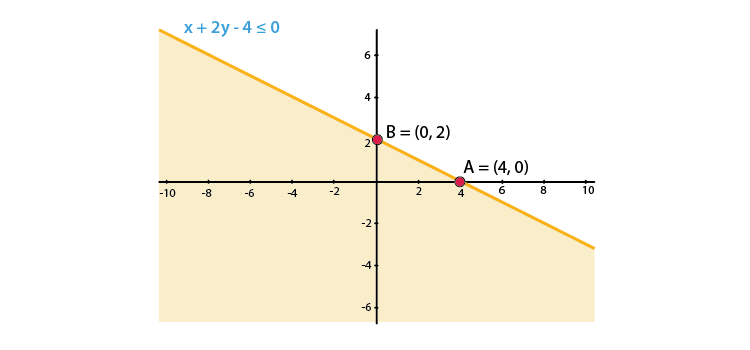2. x + 2y ≥ 6

Solution:

We shall plot the graph of the equation and shade the side containing solutions of the inequality,

You can choose any value but find the two mandatory values which are at x = 0 and y = 0, i.e., x and y–intercepts always,

x + 2y ≥ 6

So when,

 x 0 2 6 y 3 2 0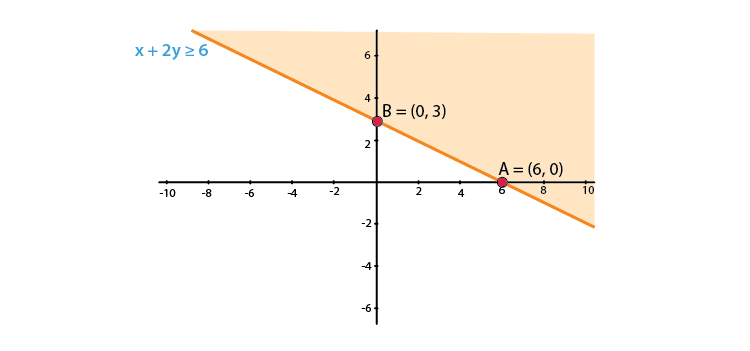3. x + 2 ≥ 0

Solution:

We shall plot the graph of the equation and shade the side containing solutions of the inequality,

You can choose any value but find the two mandatory values which are at x = 0 and y = 0, i.e., x and y–intercepts always,

x + 2 ≥ 0

x ≥ -2

As there is only one variable ‘x,’ and y = 0, which means that x has only one value when considered as an equation.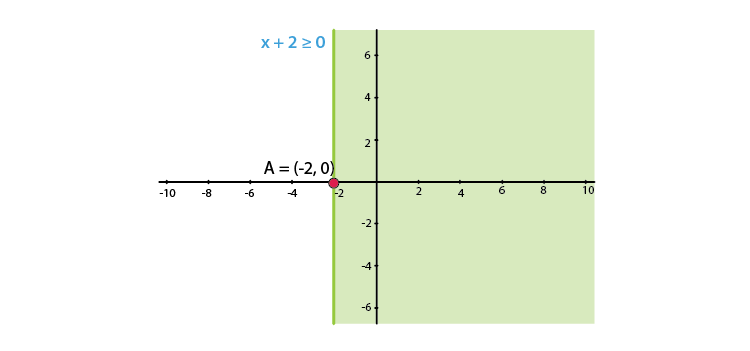4. x – 2y < 0

Solution:

We shall plot the graph of the equation and shade the side containing solutions of the inequality,

You can choose any value but find the two mandatory values which are at x = 0 and y = 0, i.e., x and y–intercepts always,

x – 2y < 0

x < 2y

So when,

 x 0 2 4 y 0 1 2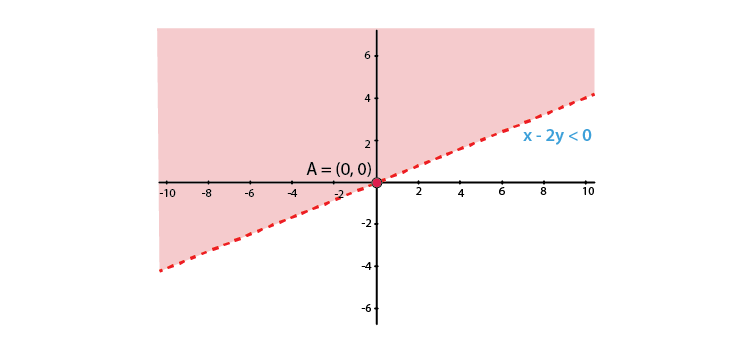5. – 3x + 2y ≤ 6

Solution:

We shall plot the graph of the equation and shade the side containing solutions of the inequality,

You can choose any value but find the two mandatory values which are at x = 0 and y = 0, i.e., x and y–intercepts always,

– 3x + 2y ≤ 6

So when,

 x 0 2 -2 y 3 6 0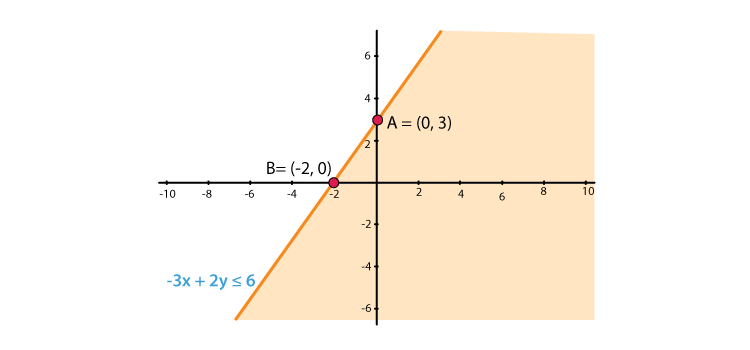EXERCISE 15.6 PAGE NO: 15.30

1. Solve the following systems of linear inequations graphically.
(i) 2x + 3y ≤ 6, 3x + 2y ≤ 6, x ≥ 0, y ≥ 0

(ii) 2x + 3y ≤ 6, x + 4y ≤ 4, x ≥ 0, y ≥ 0

(iii) x – y ≤ 1, x + 2y ≤ 8, 2x + y ≥ 2, x ≥ 0, y ≥ 0

(iv) x + y ≥ 1, 7x + 9y ≤ 63, x ≤ 6, y ≤ 5, x ≥ 0, y ≥ 0

(v) 2x + 3y ≤ 35, y ≥ 3, x ≥ 2, x ≥ 0, y ≥ 0

Solution:

(i) 2x + 3y ≤ 6, 3x + 2y ≤ 6, x ≥ 0, y ≥ 0

We shall plot the graph of the equation and shade the side containing solutions of the inequality,

You can choose any value but find the two mandatory values which are at x = 0 and y = 0, i.e., x and y–intercepts always,

2x + 3y ≤ 6

So when,

 x 0 1 3 y 2 1.33 0

3x + 2y ≤ 6

So when,

 x 0 1 2 y 3 1.5 0

x ≥ 0, y ≥ 0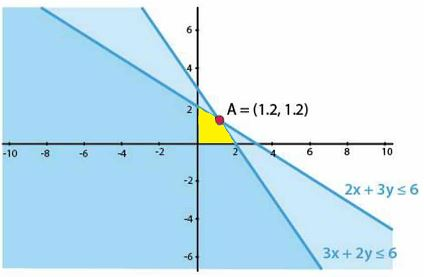(ii) 2x + 3y ≤ 6, x + 4y ≤ 4, x ≥ 0, y ≥ 0

We shall plot the graph of the equation and shade the side containing solutions of the inequality,

You can choose any value but find the two mandatory values which are at x = 0 and y = 0, i.e., x and y–intercepts always,

2x + 3y ≤ 6

So when,

 x 0 1 3 y 2 1.33 0

x + 4y ≤ 4

So when,

 x 0 2 4 y 1 0.5 0

x ≥ 0, y ≥ 0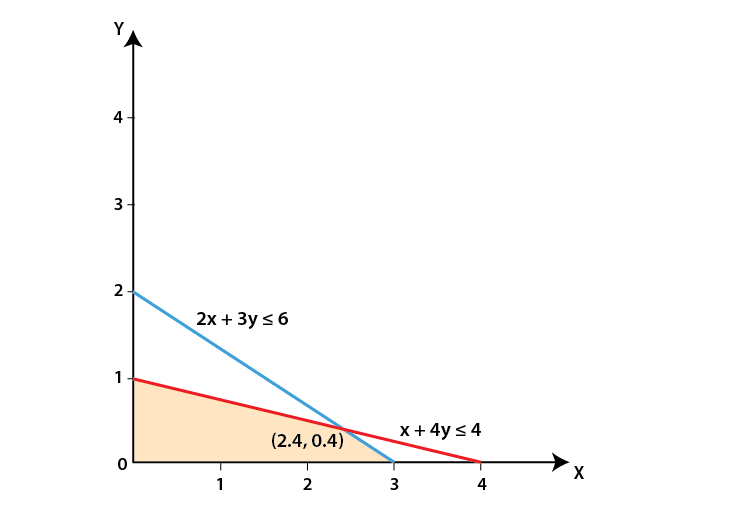(iii) x – y ≤ 1, x + 2y ≤ 8, 2x + y ≥ 2, x ≥ 0, y ≥ 0

We shall plot the graph of the equation and shade the side containing solutions of the inequality,

You can choose any value but find the two mandatory values which are at x = 0 and y = 0, i.e., x and y–intercepts always,

x – y ≤ 1

So when,

 x 0 2 1 y -1 1 0

x + 2y≤ 8

So when,

 x 0 4 8 y 4 2 0

2x + y ≥ 2

So when,

 x 0 2 1 y 2 -2 0

x ≥ 0, y ≥ 0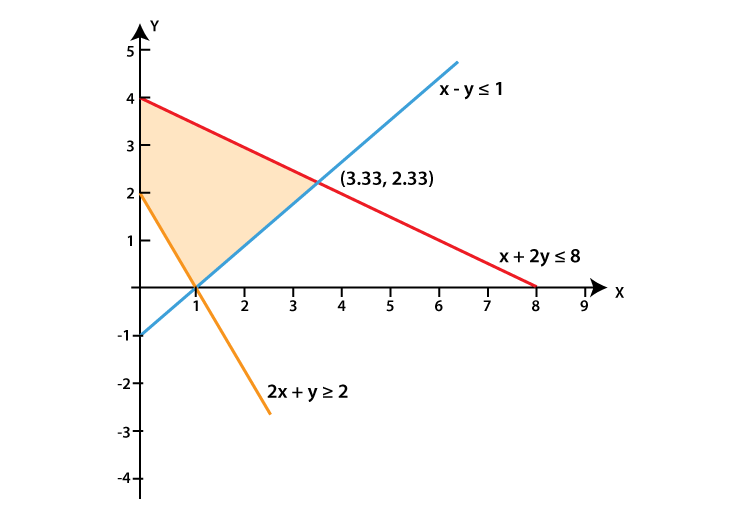(iv) x + y ≥ 1, 7x + 9y ≤ 63, x ≤ 6, y ≤ 5, x ≥ 0, y ≥ 0

We shall plot the graph of the equation and shade the side containing solutions of the inequality,

You can choose any value but find the two mandatory values which are at x = 0 and y = 0, i.e., x and y–intercepts always,

x + y ≥ 1

So when,

 x 0 2 1 y 1 -1 0

7x + 9y ≤ 63

So when,

 x 0 5 9 y 7 3.11 0

x ≤ 6, y ≤ 5 and x ≥ 0, y ≥ 0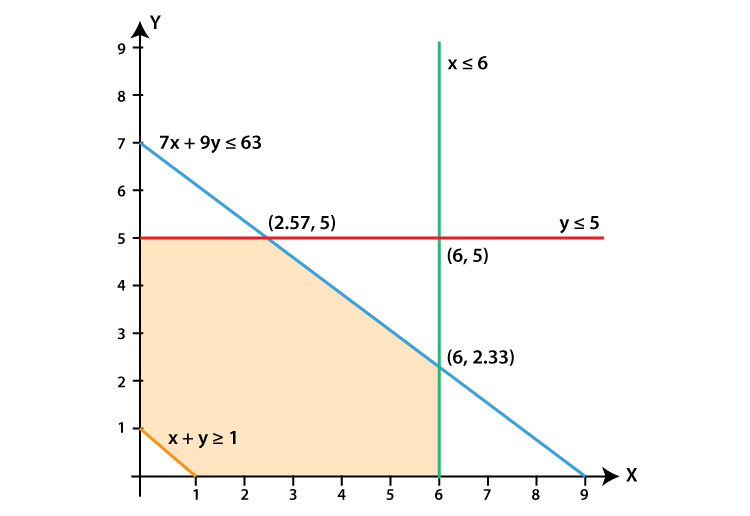(v) 2x + 3y ≤ 35, y ≥ 3, x ≥ 2, x ≥ 0, y ≥ 0

We shall plot the graph of the equation and shade the side containing solutions of the inequality,

You can choose any value but find the two mandatory values which are at x = 0 and y = 0, i.e., x and y–intercepts always,

2x + 3y ≤ 35

So when,

 x 0 5 17.5 y 11.667 8.33 0

y ≥ 3, x ≥ 2, x ≥ 0, y ≥ 0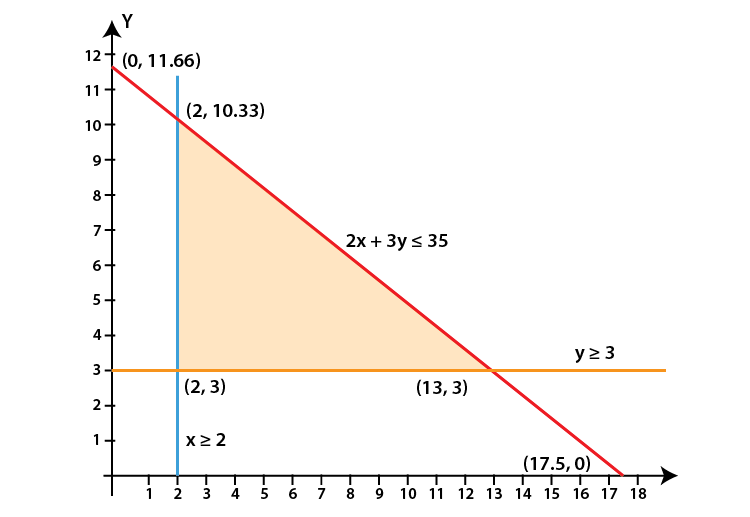2. Show that the solution set of the following linear inequations is empty set:
(i) x – 2y ≥ 0, 2x – y ≤ –2, x ≥ 0, y ≥ 0

(ii) x + 2y ≤ 3, 3x + 4y ≥ 12, y ≥ 1, x ≥ 0, y ≥ 0

Solution:

(i) x – 2y ≥ 0, 2x – y ≤ –2, x ≥ 0, y ≥ 0

We shall plot the graph of the equation and shade the side containing solutions of the inequality,

You can choose any value but find the two mandatory values which are at x = 0 and y = 0, i.e., x and y–intercepts always,

x – 2y ≥ 0

So when,

 x 0 2 4 y 0 1 2

2x – y ≤ –2

So when,

 x 0 1 -1 y 2 4 0

x ≥ 0, y ≥ 0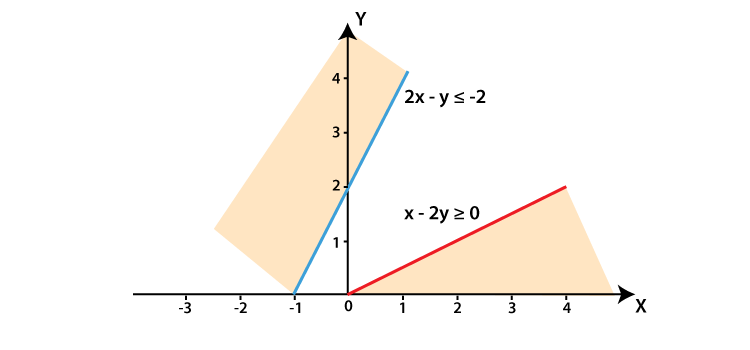The lines do not intersect each other for x ≥ 0, y ≥ 0

Hence, there is no solution for the given inequations.

(ii) x + 2y ≤ 3, 3x + 4y ≥ 12, y ≥ 1, x ≥ 0, y ≥ 0

We shall plot the graph of the equation and shade the side containing solutions of the inequality,

You can choose any value but find the two mandatory values which are at x = 0 and y = 0, i.e., x and y–intercepts always,

x + 2y ≤ 3

So when,

 x 0 1 3 y 1.5 1 0

3x + 4y ≥ 12

So when,

 x 0 2 4 y 3 1.5 0

y ≥ 1, x ≥ 0, y ≥ 0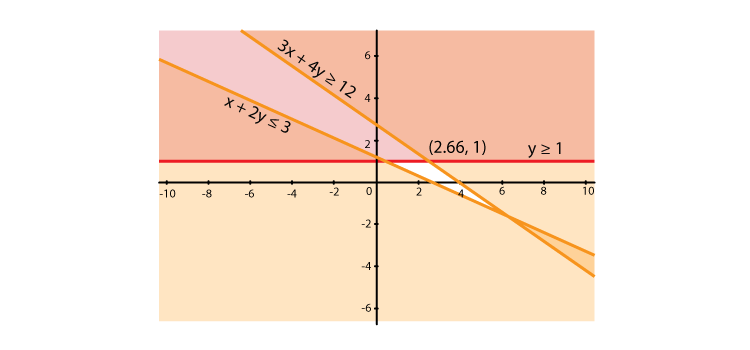3. Find the linear inequations for which the shaded area in Fig. 15.41 is the solution set. Draw the diagram of the solution set of the linear inequations.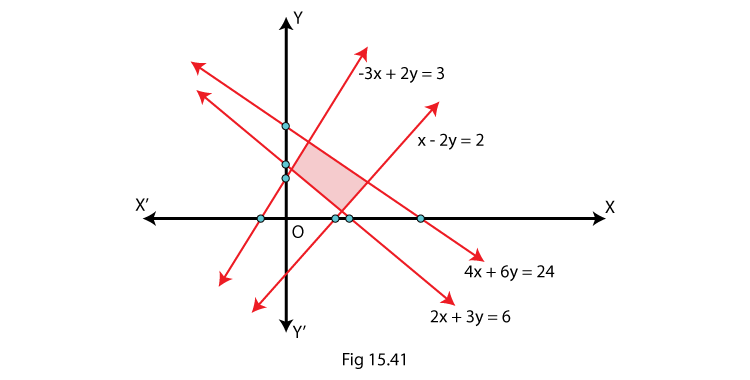Solution:

Here, we shall apply the concept of a common solution area to find the signs of inequality by using their given equations and the given common solution area (shaded part).

We know that,

If a line is in the form ax + by = c and c is positive constant. (In case of negative c, the rule becomes opposite), so there are two cases which are,

If a line is above the origin:

(i) If the shaded area is below the line then ax + by < c

(ii) If the shaded area is above the line then ax + by > c

If a line is below the origin then the rule becomes opposite.

So, according to the rules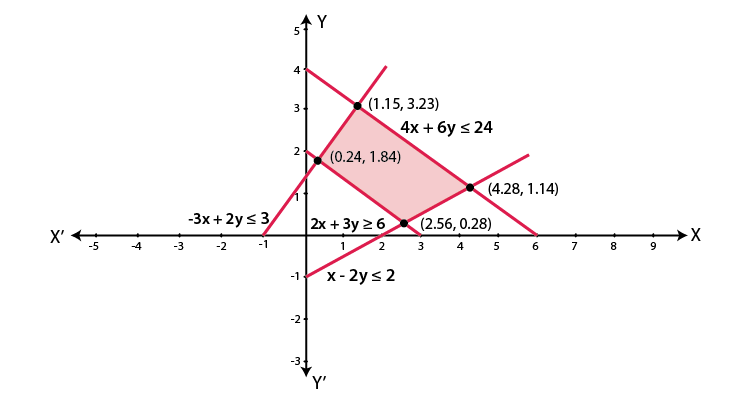4. Find the linear inequations for which the solution set is the shaded region given in Fig. 15.42.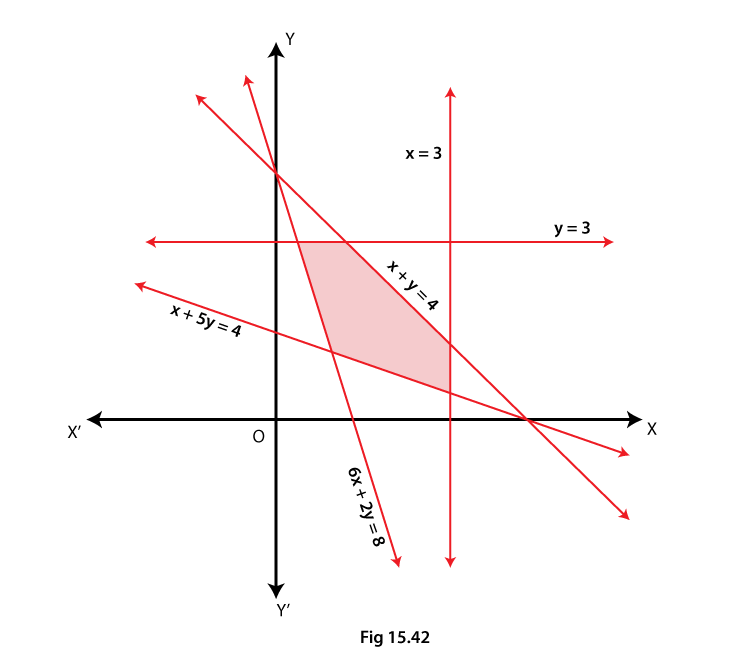Solution:

Here, we shall apply the concept of a common solution area to find the signs of inequality by using their given equations and the given common solution area (shaded part).

We know that,

If a line is in the form ax + by = c and c is positive constant.

So, according to the rules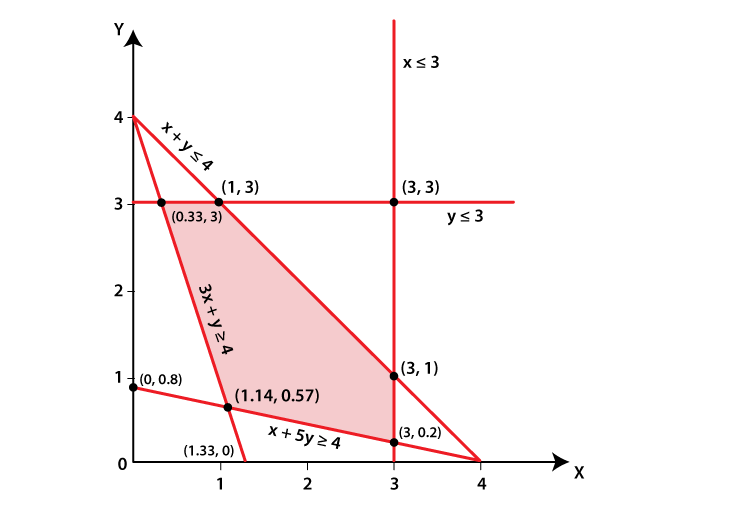### Also, access exercises of RD Sharma Solutions for Class 11 Maths Chapter 15 – Linear Inequations

Exercise 15.1 Solutions

Exercise 15.2 Solutions

Exercise 15.3 Solutions

Exercise 15.4 Solutions

Exercise 15.5 Solutions

Exercise 15.6 Solutions

## Frequently Asked Questions on RD Sharma Solutions for Class 11 Maths Chapter 15

### Why should one choose for the RD Sharma Solutions for Class 11 Maths Chapter 15 from BYJU’S?

We at BYJU’S provide 100% accurate solutions so that the students can score well in the board exams. The simple and understandable language used in explaining the topics boost confidence among students to ace the exam without fear. The solutions are framed by the team of expert faculty members after conducting a vast research on each concept. It mainly increases interest among students to learn new concepts without any difficulty. Students who have doubts while answering the textbook questions can refer to the chapter solutions to get a clear idea about the overall concepts.

### Does the BYJU’S RD Sharma Solutions for Class 11 Maths Chapter 15 provide accurate information for the board exam preparation?

Yes, the RD Sharma Solutions for Class 11 Maths Chapter 15 offered by BYJU’S is accurate enough based on the latest guidelines set by the CBSE board. The expert tutors have created the solution modules passionately so that students can learn new topics efficiently. The concepts are explained in an elaborate manner to help students grasp them without any difficulty. The solutions are detailed yet easy to comprehend making it interesting for the students to refresh the concepts after learning them.

### Where can I get the RD Sharma Solutions for Class 11 Maths Chapter 15 online?

Students can get the RD Sharma Solutions for Class 11 Maths Chapter 15 online at BYJU’S website. Students and teachers can access BYJU’S official website for RD Sharma Solutions as well which provides a wide range of study materials like text books, reference books etc. The solutions are prepared by experts with utmost care to help students understand the concepts easily and score well in their board exams.